https://dataorigami.net/blogs/napkin-folding.atom DataOrigami - Napkin Folding 2020-07-27T13:42:00-04:00 DataOrigami https://dataorigami.net/blogs/napkin-folding/highlights-from-lifelines-v0-25-0 2020-07-27T13:42:00-04:00 2020-07-27T16:43:50-04:00 Highlights from lifelines v0.25.0 Cameron Davidson-Pilon Today, the 0.25.0 release of lifelines was released. I'm very excited about some changes in this version, and want to highlight a few of them. Be sure to upgrade with:

pip install lifelines==0.25.0

Formulas everywhere!

Formulas, which should really be called Wilkinson-style notation but everyone just calls them formulas, is a lightweight-grammar for describing additive relationships. If you have used R, you'll likely be familiar with formulas. They are less common in Python, so here's an example: Writing age + salary is short-form for the expanded additive model: $$\beta_0 + \beta_1\text{age} + \beta_2\text{salary}$$. Expanding on that, the grammar allows interaction terms quite easily: age + salary + age : salary is

$$\beta_0 + \beta_1\text{age} + \beta_2\text{salary} + \beta_3 \text{age \cdot salary}$$

Actually, the previous use is so common that a short form of this entire interaction and monomial terms exists: age * salary. These are just examples, but there is a large set of transformations, ways to handle categorical variables, etc.

This is just the grammar though, and a compiler is needed to parse the string, and then translate the parsed string into code. This code can transform an initial dataset into its transformed version that allows the $$\beta$$s to be estimated. For example, transforming the following raw dataset using the formula age * salary:

df = pd.DataFrame({
'age': [35, 36, 40, 25, 55],
'salary': [60, 35, 80, 50, 100]
})

becomes:

pd.DataFrame({
'age': [35, 36, 40, 25, 55],
'salary': [60, 35, 80, 50, 100],
'age:salary': [2100, 1260, 2400, 1750, 5500]
'Intercept': [1, 1, 1, 1, 1]
})

This new dataframe can be given to any regression library to fit the $$\beta$$s. In Python, libraries like Patsy and the new Formulaic are the parser + code-generator.

Anyways, lifelines previously requested that all transformations occur in a preprocessing step, and the final dataframe given to a lifelines model. This created some problems, however:

1. The user had to learn Patsy in order to use formulas in lifelines, which is a barrier to entry.
1. In methods like plot_covariate_groups which relied on examining potentially more than one variable, the user had to manually recreate potential interaction terms or more complicated transformations.
1. Users often had to drop columns from their dataframe prior to fitting with lifelines, rather than telling lifelines what they wanted to use in the regression. This led to some ugly code.

With lifelines v0.25.0, formulas are now native (though optional) to lifelines model (old code should still work as well):

from lifelines import CoxPHFitter
cph = CoxPHFitter()
cph.fit(rossi, "week", "arrest", formula="age + fin + prio + paro * mar")
cph.print_summary(columns=['coef', 'se(coef)', '-log2(p)'])
"""
<lifelines.CoxPHFitter: fitted with 432 total observations, 318 right-censored observations>
duration col = 'week'
event col = 'arrest'
baseline estimation = breslow
number of observations = 432
number of events observed = 114
partial log-likelihood = -659.49
time fit was run = 2020-07-27 15:33:44 UTC
---
coef   se(coef)   -log2(p)
covariate
age        -0.06       0.02       8.21
fin        -0.37       0.19       4.19
prio        0.10       0.03      10.96
paro       -0.10       0.20       0.73
mar        -0.78       0.73       1.81
paro:mar    0.36       0.84       0.57
---
Concordance = 0.63
Partial AIC = 1330.98
log-likelihood ratio test = 31.78 on 6 df
-log2(p) of ll-ratio test = 15.76
"""

However, the real strength in formulas is their ability to create basis splines easily. Basis splines are highly flexible non-linear transformations of a variable - they are essential in the modern statistical inference toolkit:

cph.fit(rossi, "week", "arrest", formula="age + fin + bs(prio, df=3)")
cph.print_summary(columns=['coef', 'se(coef)', '-log2(p)'])
"""
<lifelines.CoxPHFitter: fitted with 432 total observations, 318 right-censored observations>
duration col = 'week'
event col = 'arrest'
baseline estimation = breslow
number of observations = 432
number of events observed = 114
partial log-likelihood = -659.88
time fit was run = 2020-07-27 15:36:34 UTC
---
coef   se(coef)   -log2(p)
covariate
age                -0.07       0.02       9.91
fin                -0.32       0.19       3.49
bs(prio, df=3)   1.41       0.96       2.82
bs(prio, df=3)  -0.18       1.02       0.22
bs(prio, df=3)   2.82       0.81      11.06
---
Concordance = 0.63
Partial AIC = 1329.76
log-likelihood ratio test = 31.00 on 5 df
-log2(p) of ll-ratio test = 16.70
"""

Importantly, the new transform logic in lifelines is extended to the predict and plotting methods too.

For models that have more than one parameter, like WeibullAFTFitter , formulas can be crafted for each parameter.

from lifelines import WeibullAFTFitter
wf = WeibullAFTFitter()
wf.fit(rossi, "week", "arrest", formula="age + fin + paro * mar", ancillary="age * fin")
wf.print_summary(columns=['coef', 'se(coef)', '-log2(p)'])
"""
<lifelines.WeibullAFTFitter: fitted with 432 total observations, 318 right-censored observations>
duration col = 'week'
event col = 'arrest'
number of observations = 432
number of events observed = 114
log-likelihood = -681.82
time fit was run = 2020-07-27 16:49:31 UTC
---
coef   se(coef)   -log2(p)
param   covariate
lambda_ Intercept   2.19       0.68       9.59
age         0.11       0.03      10.13
fin         0.12       0.19       0.97
paro        0.17       0.14       2.19
mar         0.33       0.53       0.93
paro:mar   -0.14       0.61       0.29
rho_    Intercept   1.36       0.41       9.98
age        -0.05       0.02       7.25
fin        -0.38       0.54       1.04
age:fin     0.02       0.02       1.74
---
Concordance = 0.62
AIC = 1383.65
log-likelihood ratio test = 29.60 on 8 df
-log2(p) of ll-ratio test = 11.97
"""

New KMunicate plots!

One of my favourite types of research articles are on changes to improving scientific understanding and communication. Last year, a paper, by T. Morris et al., came out that surveyed statisticians, clinicians and stakeholders on how to better communicate the workhorse of survival analysis, Kaplan-Meier (KM) curves. A number of potential options were shown to the over 1100 survey participants, and ratings to each option were made. The survey results show two changes that could be made to improve understanding of KM curves.

1. Always show confidence intervals around the curves (lifelines does this by default)

2. Present all the summary information at the bottom. Many KM curves would present at-risk numbers, and lifelines had this option as well:But the participants really liked all summary information, including deaths and censorship, not just at-risk. lifelines now presents all this information: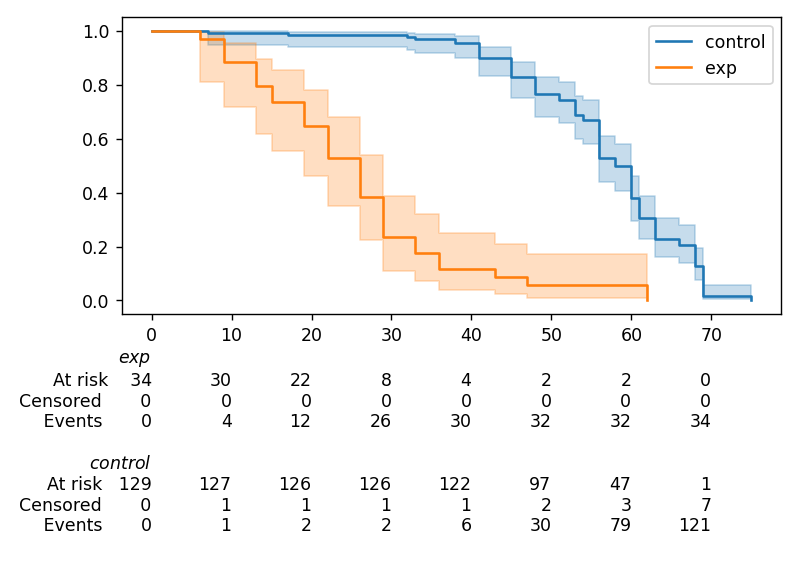It's not displayed by default (that may change), but with the at_risk_counts kwarg in the call to KaplanMeierFitter.plot.

This small change has the potential to be a massive improvement in understanding these plots. I'm so happy I saw this paper come across my desk.

Performance improvements

Performance improvements was actually part of a release a few weeks back and not the v0.25.0, but I wanted to highlight it anyways. I found a bug in the switching algorithm that we use for choosing which algorithm to run for the Cox model (see post on how that's done here). This bug made the choice of algorithm sub-optimal, specifically for very large datasets. After fixing the bug, CoxPHFitter is able to process millions of rows in less than a tenth of a second (not to trash on it, but just for comparison: R takes on the order of seconds, and the core algorithm is written in C). This is probably one of the fastest Cox-Efron models, and it's written only in Python. This makes me really proud.

Conclusion

I'm really happy with where this release landed. There were lots of ups and downs in code quality and structure, but I feel like I settled on something nice. Formulas are a big deal, and will take lifelines to the next level. Future release will have support for partial-effect plots, and more.

You can see all the improvements, and important API changes, in v0.25.0 here.

]]>
https://dataorigami.net/blogs/napkin-folding/an-l1-2-penalty-in-cox-regression 2020-07-17T11:27:00-04:00 2020-07-17T21:35:30-04:00 An L½ penalty in Cox Regression Cameron Davidson-Pilon Following up from a previous blog post where we explored how to implement an $$L_1$$ and elastic net penalty to induce sparsity, a paper, by Xu Z B, Zhang H, Wang Y, et al., explores what a $$L_{1/2}$$ penalty is and how to implement it.

But first, I think we are familiar with an $$L_1$$ penalty, but what is an $$L_0$$ penalty then? If you work out the math, it is a penalty that counts the number of non-zero coefficients, independent of the magnitude of the coefficients:

$$ll^*(\theta, x) = \sum_i^N ll(\theta, x_i) - \lambda \sum_{k=0}^D 1_{\theta_k \ne 0}$$

where $$D$$ is the number of potential parameters. Thinking about this for a moment, this means that the $$L_0$$ penalty minimizes the AIC, since the AIC is:

$$AIC = -2 ll + 2D^*$$

where $$D^*$$ is the number of parameters in the model. It turns out that $$L_0$$ penalties encourage lots of sparsity in their solutions, much more than $$L_1$$.

Given that, the $$L_{1/2}$$ penalty is the balance between penalizing the magnitudes of the coefficients and encouraging lots of sparsity. The paper linked above gives reasons why $$L_{1/2}$$ is perhaps superior to both $$L_1$$ and $$L_0$$. Importantly, solving $$L_0$$ is NP-hard because it involves a combinatorial explosion of potential solutions that can't be solved with gradient methods.

The authors provide a very simple algorithm for solving the $$L_{1/2}$$ problem, see Section 3 of the paper. It involves repeatedly solving a related $$L_1$$ problem with updating coefficient-specific penalizer values. In lifelines, we recently introduced the ability to set specific coefficient penalizer values, and we can solve $$L_1$$ problems too. Let's see if we can solve $$L_{1/2}$$ problems now:

def l_one_half_cox(lambda_, df, T, E):
EPSILON = 0.00001
weights = lambda_ * np.ones(df.shape-2)
cph = CoxPHFitter(l1_ratio=1.0, penalizer=weights)
cph.fit(rossi, "week", "arrest")
max_iter = 20
i = 1
while i < max_iter:
weights = lambda_ / (np.sqrt((cph.params_.abs()).values) + EPSILON)
cph = CoxPHFitter(l1_ratio=1.0, penalizer=weights)
cph.fit(rossi, "week", "arrest")
i += 1
return cph.params_

In the above code, we repeatedly solve a new $$L_1$$ problem with updated penalizer weights. This, according to the authors and my own assumption that it can be extended easily to the Cox model, gives us our $$L_{1/2}$$ solution. Graphically, we can vary the lambda_ parameter can see how the coefficient solutions change: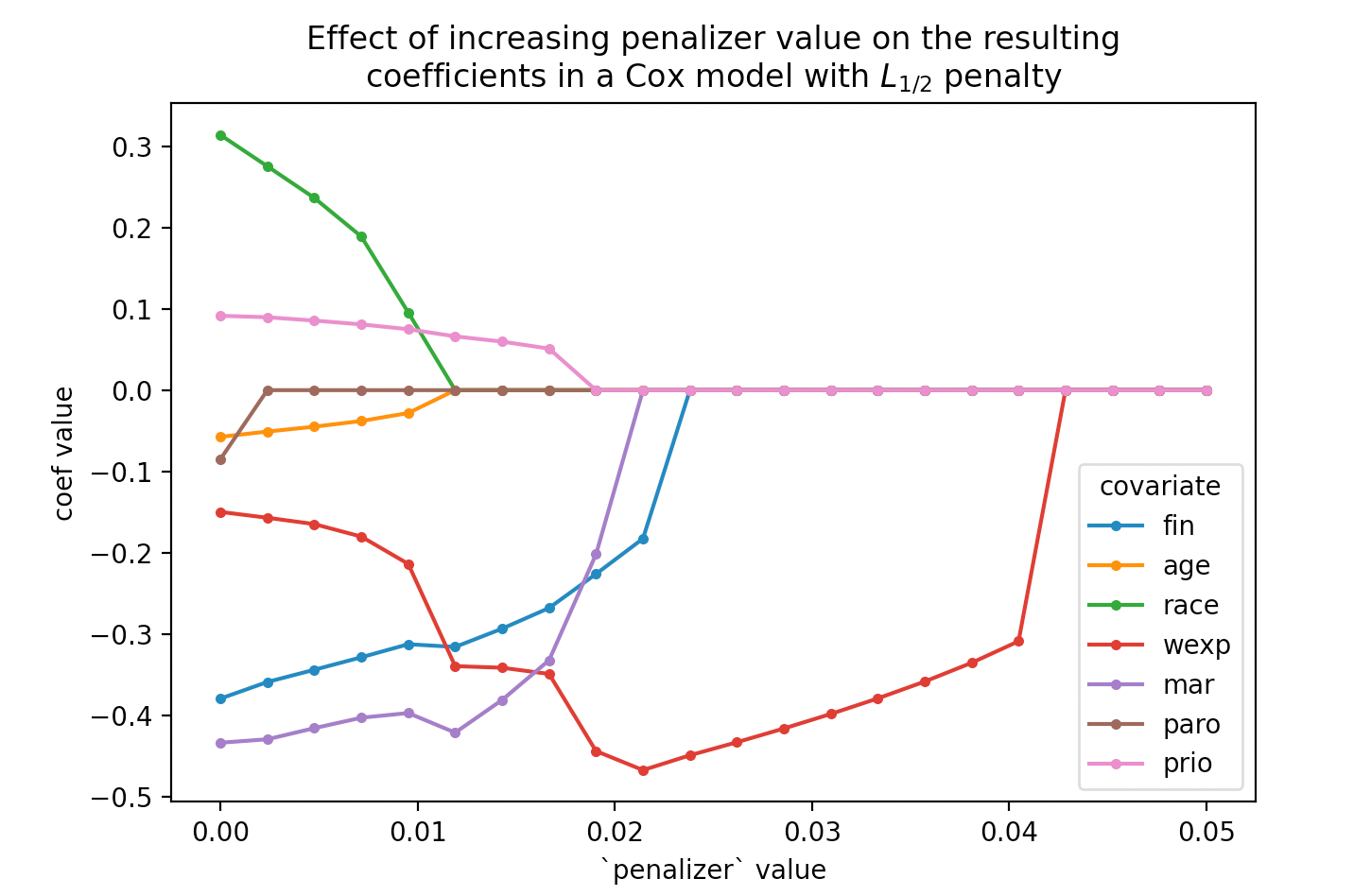Compare this to our $$L_1$$ solution: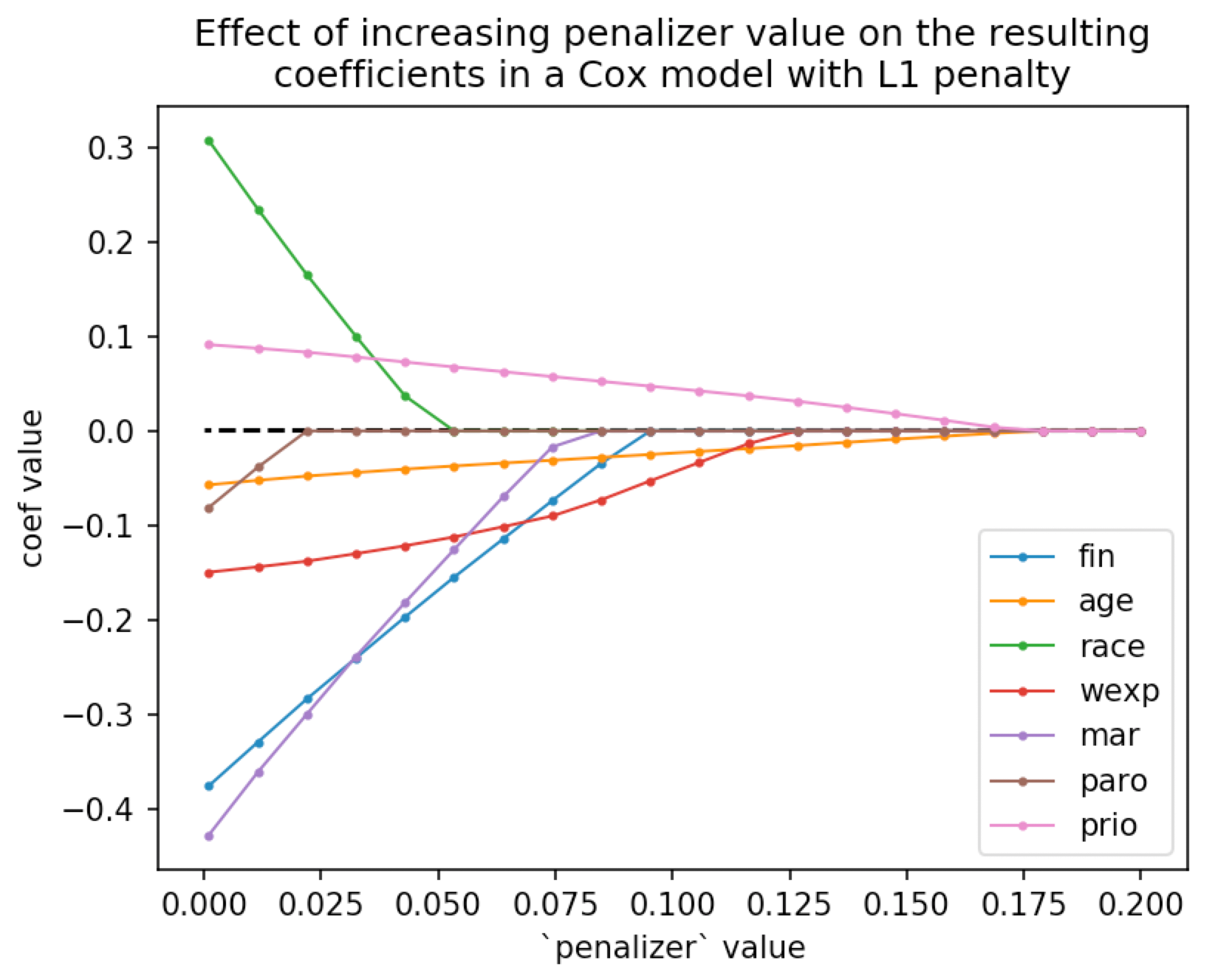]]>
https://dataorigami.net/blogs/napkin-folding/an-accelerated-lifetime-spline-model 2020-06-15T20:22:00-04:00 2020-07-17T21:38:58-04:00 An accelerated lifetime spline model Cameron Davidson-Pilon A paper came out recently with a novel accelerated lifetime (AFT) model with cubic splines. This should pique your interest for a few reasons:

1. It helps dethrone the Proportional Hazard (PH) model as the default survival model. People like the PH model because it doesn't make any distributional assumptions. However, like a Trojan horse, there are very strong implicit assumptions that are inherited, often which are too restricting. Suffice to say, I am not a big proponent of the PH model.

2. A spline-based AFT model weakens the CPH's throne because the model can fit to a larger space of potential models. For example, the Weibull AFT model is a special case of this new AFT model. The authors of the paper also carefully demonstrate that it often has lower bias, standard error, or AIC than other popular AFT models (Generalized Gamma, Generalized F).

3. AFT models are just simpler to explain. Coefficients of AFT models have a much nicer interpretation than PH or Proportional Odds (PO) models. Simply: a positive (negative) coefficient multiplicatively accelerates (decelerates) a subject's time-to-event. So, a coefficient of 2 means that a subject experiences the event twice as fast as a baseline subject, on average.

I'm too lazy to give the mathematical details of the model (just drank a strong beer), but what I do want to mention is that the model is implementable in lifelines using our custom model syntax. Here's the code

Update: it's now part of lifelines as lifelines.CRCSplineFitter. Happy coding!

]]>
https://dataorigami.net/blogs/napkin-folding/new-sibling-blog-on-food-science-fermentation-and-statistics-controlledmold 2020-05-28T08:20:00-04:00 2020-05-28T08:20:51-04:00 New sibling blog on food science, fermentation, and statistics: ControlledMold Cameron Davidson-Pilon I've started a new blog at ControlledMold that is more about the food, fermentation and cell-ag side of my research. There are still data science and statistics articles, too, like Using a Secchi Stick to Measure Cell Density. Enjoy!

]]>
https://dataorigami.net/blogs/napkin-folding/controlling-bacterial-growth-in-fermentation-with-hurdle-technology-and-survival-analysis 2020-04-12T11:33:00-04:00 2020-04-12T11:52:32-04:00 Controlling bacterial growth in fermentation with hurdle technology and survival analysis Cameron Davidson-Pilon This article is a nice intersection of some of the topics I've been thinking about lately: bacteria, food, and survival analysis, and part of a larger project I've been working on (stay tuned).

The bacteria C. Botulinum is responsible for creating one of the most dangerous chemicals known to man: botulinum toxin. If ingested, incredibly small amounts of this toxin can kill even a healthy person. Thankfully, food scientists and microbiologists have developed ways to control C. Botulinum. Any of the application of high acidity, high salinity, low/high temperature, oxygen-exposure can slow the growth of the bacteria, and in extreme amounts, destroy the bacteria. However, for food purposes, it is sufficient to slow the growth of the bacteria, typically by extending its lag period to an extremely long time. The lag period is a phase where a microorganism becomes accustomed to its new environment, before starting cell division (and entering the exponential phase of growth) - see figure below. For example, the reason why vinegar is used in home canning is to create an environment that is unfavourable (too acidic) for any remaining microbes (and there always are some) won't start multiplying. Vinegar isn't necessarily killing the bacteria, just extending its lag phase. Improper acidification in home canning is actually the cause of most botulism outbreaks in the Western world.Hurdle Technology

The idea of hurdle technology is to create more than one unfavourable growth condition for bacteria (or their spores). This idea is that either there is a super-additive negative effect on the growth, or that one condition can be "relaxed" to improve sensory characteristics (ex: less vinegar, but more salt) and still achieve the desired control.

Hurdle technology is present in almost all foods, typically disguised as some preservation technique. Consider cheese: it has low water activity (moisture), stored at cool temperatures, and high acidity. All of these conditions are hurdles.

Fermentation

One concern that I often see beginner fermenters worry about is botulism. This is understandable: it's somewhat uncomfortable leaving a jar of vegetables out of the fridge for weeks, and then eating it. This goes against everything we have been taught about food safety. And though rare, botulism is deadly enough that the expected risk is high enough to cause worry.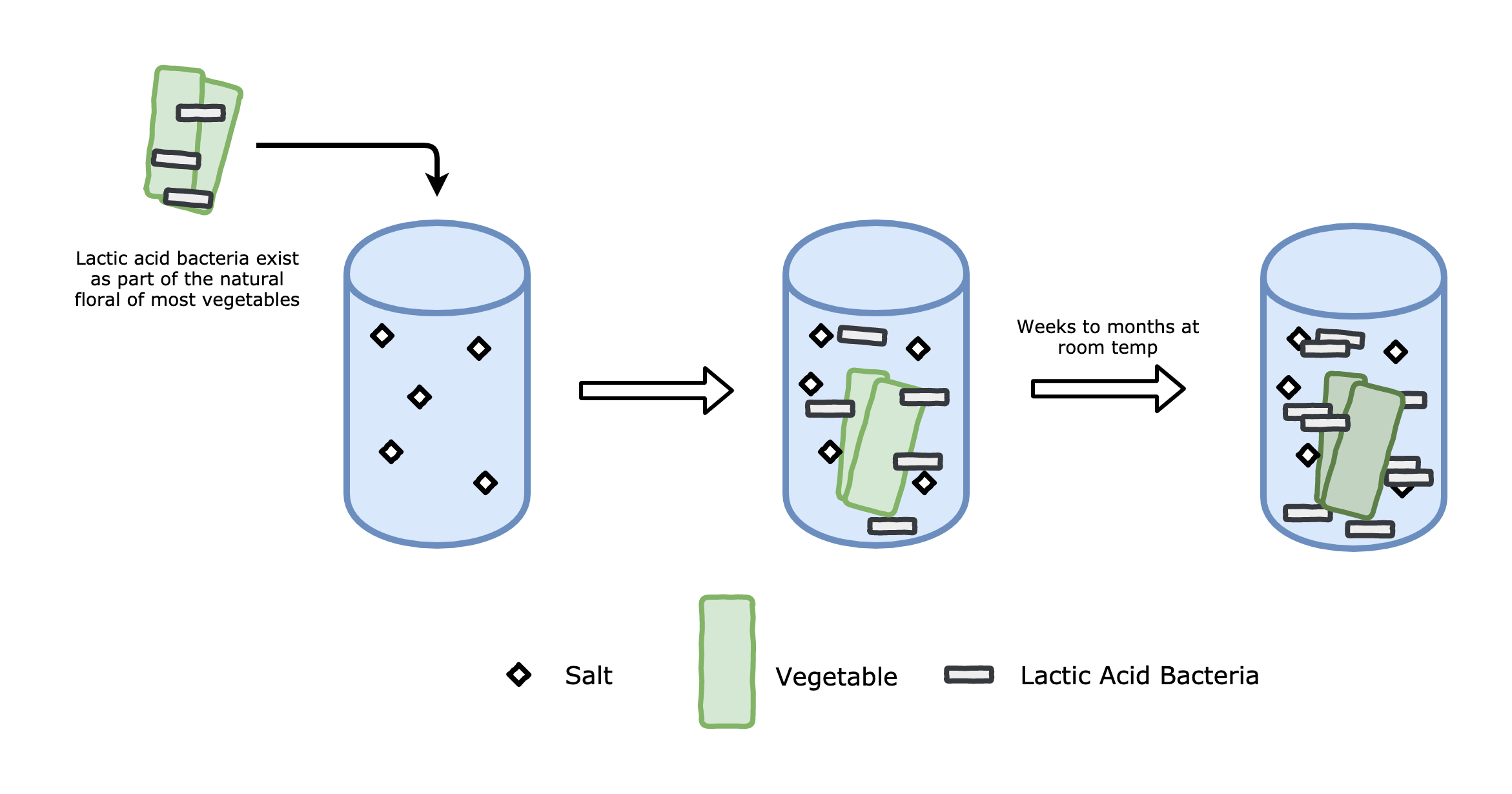However, hurdle technology is at play here. The idea is to use multiple hurdles, in this case salt, acid, and enough competing microbes, to effectively pause any botulism bacteria in their lag period. The salt is added in the brine, and the acid can be provided by the lactic acid bacteria or manually added to the brine. For brines below 6% NaCl, which almost all fermentation brines are, the lactic acid bacteria have essentially no lag period and very quickly enter their exponential phase . However, C. Botulinum can survive moderate amounts of salt as well, and if present, could also start multiplying. What we'd like is to create an environment that extends the lag period of C. Botulinum far enough such that the lactic acid bacteria can completely out compete any C. Botulinum present. Let's get some data.

The data comes from a 1983 paper on the effects of salt concentration and pH on C. Botulinum . Below is a screenshot of the conditions of the trials (first and second columns) and observed lag period (third column).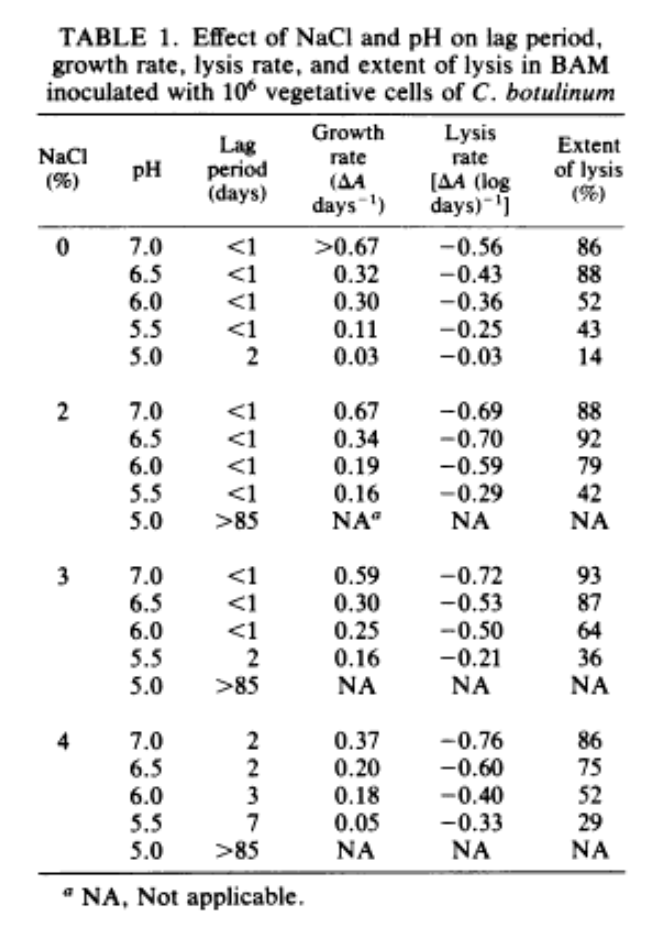We can see that we actually don't have many exact observations. Most observations are <1, meaning that the authors didn't observe the lag period end exactly, but instead noted that it happened sometime within the first day. Similarly, for some pH and salt concentrations, the lag period went past the authors' timeline of 85 days, so they recorded this as >85. This type of data is considered censored, so let's use survival analysis to analyze the relationship between pH, salt and lag periods.

Boot up Python

We are interested in determining the survival distribution of the lag period, conditional on pH and salt concentration. Don't get confused about the "survival" part here: we are not measuring survival of bacteria - we are using survival analysis, a technique for duration data, to model how long the bacteria is in its lag period. We have data that is both right-censored (>85) and left-censored (<1). We can encode this data by using two columns, one for a lower bound (which may be 0) and one for an upper bound (which may be infinity). For exact measurements, the lower and upper bound are equal:

print(df)
NaCl %   pH  lower_bound_days  upper_bound_days
0        0  7.0               0.0               1.0
1        0  6.5               0.0               1.0
2        0  6.0               0.0               1.0
3        0  5.5               0.0               1.0
4        0  5.0               2.0               2.0
5        2  7.0               0.0               1.0
6        2  6.5               0.0               1.0
7        2  6.0               0.0               1.0
8        2  5.5               0.0               1.0
9        2  5.0              85.0               inf
10       3  7.0               0.0               1.0
11       3  6.5               0.0               1.0
12       3  6.0               0.0               1.0
13       3  5.5               2.0               2.0
14       3  5.0              85.0               inf
15       4  7.0               2.0               2.0
16       4  6.5               2.0               2.0
17       4  6.0               3.0               3.0
18       4  5.5               7.0               7.0
19       4  5.0              85.0               inf
20       6  7.0              85.0               inf
21       6  6.5              85.0               inf
22       6  6.0              85.0               inf
23       6  5.5              85.0               inf
24       6  5.0              85.0               inf

Let's use a Weibull survival regression model. That is, our functional form looks like:

\begin{align}&P(\text{lag period} > t) \\ &= S(t\;|\;\text{pH},\text{salt%})\\ &= \exp{\left(-\left(\frac{t}{\lambda(\text{pH, salt%})}\right)^\rho\right)} \end{align}

where

$$\lambda(\text{pH, salt%}) =\exp{(\beta_1\text{pH} + \beta_2\text{salt%} + \beta_0)}$$

The coefficients and $$\rho$$ are to be estimated from the data. Fitting is done in lifelines:

from lifelines import *
aft = WeibullAFTFitter()
aft.fit_interval_censoring(
df,
lower_bound_col="lower_bound_days",
upper_bound_col="upper_bound_days")
aft.print_summary()
"""
<lifelines.WeibullAFTFitter: fitted with 25 total observations, 19 interval-censored observations>
lower bound col = 'lower_bound_days'
upper bound col = 'upper_bound_days'
number of observations = 25
number of events observed = 6
log-likelihood = -25.70
time fit was run = 2020-04-12 13:20:30 UTC
---
coef  exp(coef)   se(coef)   coef lower 95%   coef upper 95%  exp(coef) lower 95%  exp(coef) upper 95%
lambda_ NaCl %       2.51      12.27       0.69             1.16             3.86                 3.18                47.43
pH          -4.87       0.01       1.48            -7.77            -1.98                 0.00                 0.14
_intercept  23.38   1.43e+10       7.16             9.35            37.41             11520.08             1.77e+16
rho_    _intercept  -0.73       0.48       0.35            -1.41            -0.05                 0.24                 0.95
z      p   -log2(p)
lambda_ NaCl %      3.64 <0.005      11.81
pH         -3.30 <0.005      10.01
_intercept  3.27 <0.005       9.84
rho_    _intercept -2.11   0.04       4.83
---
Log-likelihood ratio test = 31.94 on 2 df, -log2(p)=23.04

Looking at the coef column above, we can see that lower pH increases the lag period and a higher salt concentration increases the lag period. If we suspect there to be super-additive effects between salt and pH, we can try adding an interaction term. After doing so, the fit isn't very good, so we leave it out.

Aside: I found a handful of bugs (convergence errors, API mistakes) when doing this project, which is great, because it makes lifelines more robust for other users.

Now that we can connect pH and salt concentration to "probability of botulism growth", we can build a rule that minimizes a fermentation's risk to botulism. Specifically, if we provide the lactic acid bacteria (which has no lag period) a sufficient head start, we can rest assured they will out compete the bad bacteria and further acidify the environment. Let's say we want the probability of botulism growth in the first 6 hours to be less than 1%, which is more than enough time to give good bacteria a head start. In math:

\begin{align} & P(\text{lag period} < 0.25) < 0.01\\ & \iff P(\text{lag period} > 0.25) > 0.99 \\ & \iff S(0.25) > 0.99 \\ & \iff S(0.25) = \exp{\left(-\left(\frac{0.25}{\lambda(\text{pH, salt%})}\right)^\rho\right)} > 0.99 \\ & \iff \exp{\left(-\left(\frac{0.25}{\exp{(2.51 \cdot \text{salt%} -4.87 \cdot \text{pH} + 23.38)}}\right)^{0.48}\right)} > 0.99 \\ \end{align}

Performing some algebra, and some rounding to make the final formula nicer, the above inequality is satisfied if:

$$2 \cdot \text{pH} - \text{salt%} < 9$$

If your initial brine satisfies this, you can be quite certain that the risk of botulism is nil. For example, if you want to decrease salt concentration by a percent, you should lower the pH by 1/2 a unit. Keep in mind, this is for a very conservative application, and even if this inequality is not satisfied, this does not mean that botulism will develop. This formula can be used for the most worried of individuals. There are of course many other ways to reduce the risk of botulism, but that's for a non-Data Origami article!

]]>
https://dataorigami.net/blogs/napkin-folding/l1-penalty-in-cox-regression 2020-03-09T09:32:00-04:00 2020-07-17T15:17:02-04:00 L₁ Penalty in Cox Regression Cameron Davidson-Pilon

In the 00's, L1 penalties were all the rage in statistics and machine learning. Since they induced sparsity in fitted parameters, they were used as a variable selection method. Today, with some advanced models having tens of billions of parameters, sparsity isn't as useful, and the L1 penalty has dropped out of fashion.

However, most teams aren't using billion parameter models, and smart data scientists work with simple models initially. Below is how we implemented an L1 penalty in the Cox regression model.

Smoothing the absolute value

The log-likelihood we wish to maximize looks like:

$$ll^*(\theta, x) = \sum_i^N ll(\theta, x_i) - \lambda||\theta||_1$$

where $$||\cdot ||_1$$ is the sum of absolute values of the parameter vector $$\theta$$. With L2 penalties, the penalty term is differentiable, and we can easily find the gradient w.r.t. the parameter vector, which enables us to use iterative solvers to solve this optimization problem. However, when our penalty is L1, we don't have a smooth derivative.

We solve this by replacing $$||\cdot ||_1$$ with a smooth version:

$$\text{softabs}(\theta, a) = \frac{1}{a} \log(1 + \exp(-a\theta)) + \frac{1}{a}\log(1 + \exp(a\theta))$$

As $$a→\inf$$, this converges to the absolute value of $$\theta$$. This smooth absolute value is differentiable everywhere too. With that in mind, we can use our iterative solvers again, and increase $$a$$ each iteration. I don't think there is any simple solution to choose $$a$$, but I've found that increasing it exponentially works well.

Elastic net penalty

We can combine this L1 penalty with an L2 penalty to get the elastic-net penalty, introduce a new parameter $$\rho$$ to control weighting between the two:

$$ll^*(\theta, x, a) = \sum_i^N ll(\theta, x_i) - \lambda(\rho||\theta||_2^2 + (1-\rho) \text{softabs}(\theta, a))$$

And remember: cool kids don't take derivatives by hand! Why waste time and make mistakes trying to compute the first and second derivative of this penalty - let autograd do it!

from autograd import numpy as anp
def soft_abs(x, a):
return 1 / a * (anp.logaddexp(0, -a * x) + anp.logaddexp(0, a * x))
def penalizer(beta, a, lambda_, l1_ratio):
return lambda_ * (l1_ratio * soft_abs(beta, a).sum() + (1 - l1_ratio) * (beta ** 2).sum()) d_penalizer = elementwise_grad(penalizer) dd_penalizer = elementwise_grad(elementwise_grad(penalizer) i = 0 while converging: i += 1 h, g, ll = get_gradients(X, beta) ll -= penalizer(beta, 1.3 ** i, 0.1, 0.5) g -= d_penalizer(beta, 1.3 ** i, 0.1, 0.5) h[np.diag_indices(d)] -= dd_penalizer(beta, 1.3 ** i, 0.1, 0.5) # update beta and converging logic! Not shown here.

Example in Cox model

In lifelines 0.24+, we introduced the L1 penalty for Cox models. We can visualize the sparsity effect of the L1 penalty as we increase the penalizer term:

from lifelines import CoxPHFitter
results = {}
for p in np.linspace(0.001, 0.2, 40):
cph = CoxPHFitter(l1_ratio=1., penalizer=p).fit(rossi, "week", "arrest")
results[p] = cph.params_
pd.DataFrame(results).T.plot()]]>
https://dataorigami.net/blogs/napkin-folding/non-parametric-survival-function-prediction 2019-08-27T21:32:00-04:00 2019-10-23T10:44:19-04:00 Non-parametric survival function prediction Cameron Davidson-Pilon

As I was developing lifelines, I kept having a feeling that I was gradually moving the library towards prediction tasks. lifelines is great for regression models and fitting survival distributions, but as I was adding more and more flexible parametric models, I realized that I really wanted a model that would predict the survival function — and I didn't care how. This led me to the idea to use a neural net with $$n$$ outputs, one output for each parameter in the cumulative hazard, $$H(t)$$. lifelines has implemented the linear version of this, where each parameter in the cumulative hazard is a linear function of the variables:

Linear:

$$H(t\;|\;x) = f(a, b, c) \\a = a(x \cdot \beta_1^T) \\b = b(x \cdot \beta_2^T) \\c = c(x \cdot \mathbf{\beta}_3^T)$$

Neural Net (NN):

$$H(t\;|\;x) = f(a, b, c) \\a = \text{NN}(x)_1 \\b = \text{NN}(x)_2 \\c = \text{NN}(x)_3$$

If my NN has no hidden layer, it's equivalent to the linear case. Great, so using a NN and a function $$f$$ provides us a good way to predict survival functions. Can we do better? Well, we are stuck with a particular $$f$$, which may or may not be flexible enough to capture all the nuances of possible survival. For example, SaaS churn has very unique survival behavior that a traditional parametric model couldn't capture well.

Thinking more about that blog post linked above, I realized that I could use a piecewise approach and split my timescale into partitions as fine as I want, and then predict a constant hazard in each interval. Graphically, something like this: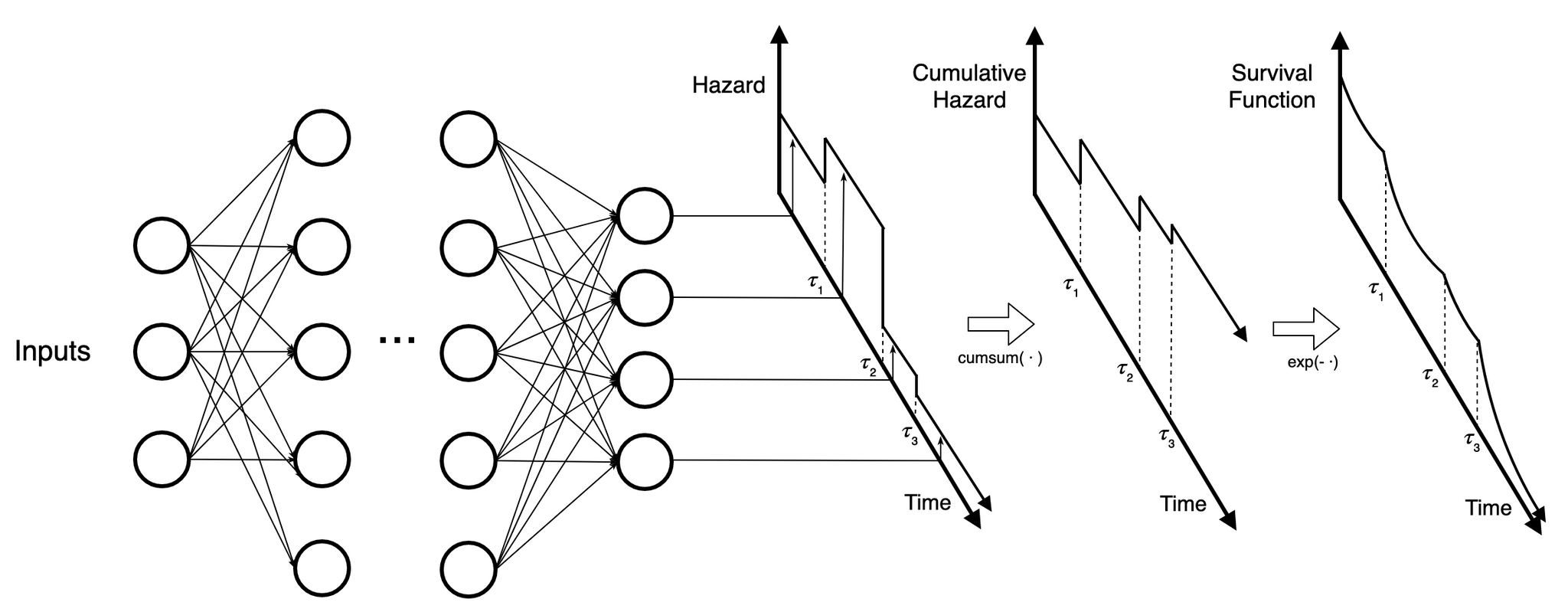Each output predicts the hazard in a time interval. This piecewise constant hazard can form the cumulative hazard, and then the survival function.

As the NN increases in depth and complexity, each output benefits from the information in the other time periods. For example, if the model thinks that a certain generated feature is really important, it's exposed for all outputs to use.

What about those taus — which I call breakpoints — how are they determined? There are a few options. The first option is the manually specify them. This is okay if we have apriori knowledge of important times, but often we don't. A second option is to expand the neural net to return breakpoints as well. Unfortunately I haven't gotten this model to converge well. The final option considered is to use the dataset to choose breakpoints. In the Kaplan Meier model, the time points of change in the survival function are equal to the unique event times. More generally, the density of the changes in survival is proportional to the density of observed event times. Using this, we can programmatically choose good breakpoints by first creating a density estimate of observed event times, and spacing out breakpoints in proportion to high density. That is, we solve $$p=F_T(t)$$ for $$t$$ at evenly spaced $$p$$ values. Below is an example CDF and how breakpoints are determined: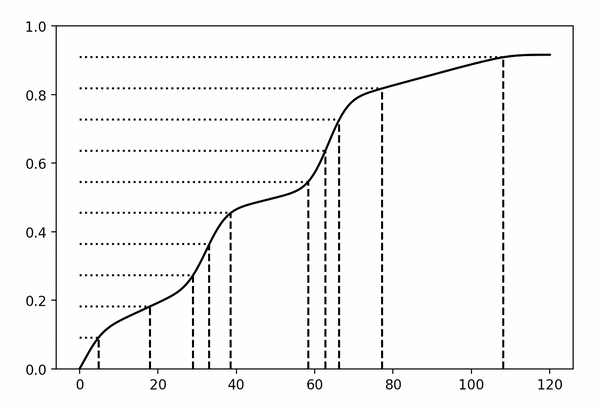The number of breakpoints is more arbitrary, and can be manually set or heuristically set (like proportional to the square root the number of observed event times).

Implementation in Python

So, to recap: we choose breakpoints in proportion to density of event times (which is where the most information is), and use a neural net to determine a subject's hazard in that interval. This is exactly what my new Python library, implements. At the moment, I am building upon the computational library Jax. lifelike's API is similar to Keras, and users familiar with Keras (and Jax) could jump in immediately. The library is also quite opinionated, and based on my own philosophy on survival analysis. For example:

1. The predicted survival function is returned, and no effort is made to compute a point estimate. This aligns with my belief that a distribution has enormous advantages (and on the other side of that coin: point estimates are overrated). Users can compute their own points estimates if they wish

2. Fitting is done against the log-likelihood loss. The survival analysis log-likelihood contains all the information around censoring and observed event times. Is it interpretable? Not really, but most losses are not anyways. The goal is to minimize loss, and I believe the log-likelihood is a terrific loss.

Let's see some inference done with lifelike. The model was trained on an artificial SaaS churn dataset. No prior knowledge about the breakpoints were provided. Below we see the predicted survival function and predicted hazard of a subject.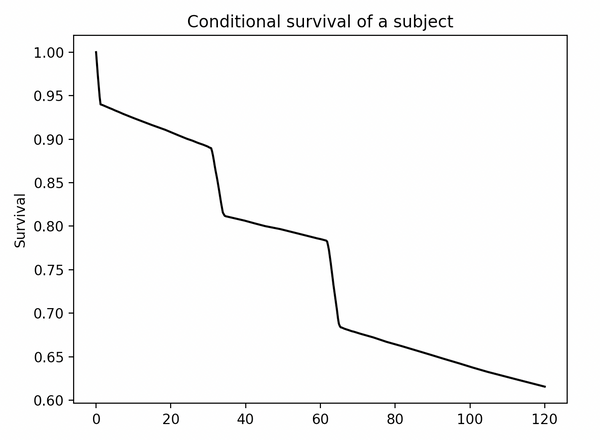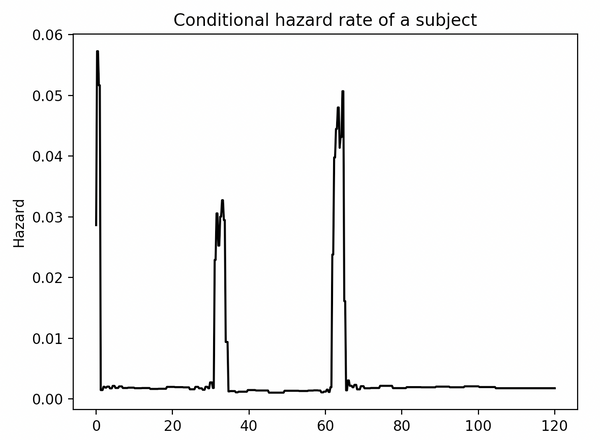The model was correctly able to find the periods of high SaaS customer churn, and predict the subject's survival function.

Conclusion

This NN piecewise-constant model isn't new, and has been published recently in literature before. However, almost all the literature has a very shallow network, and is fit using BFGS. lifelike is the first implementation in a modern computational graph framework, and leaning on Jax means we get autodiff, JIT and GPUs for free. We can scale this model is any dataset size or network we wish (limited only by Jax). For example, my upcoming research could be using images from spectrometers as an input to NNs — lifelike theoretically can handle that.

I hope this blog article inspired you or gave you some new ideas. lifelike is very experimental, and I'm still iterating on APIs and functionality, so use at your own risk. Stay tuned for more updates!

]]>
https://dataorigami.net/blogs/napkin-folding/bayesian-cell-counts-pt-2 2019-05-22T22:31:00-04:00 2019-05-23T14:01:02-04:00 Bayesian cell counting Pt. 2 - Growth over time Cameron Davidson-Pilon

I’ve started growing yeast in my closet-turned-laboratory. There’s a reason why I am growing yeast, but that’ll be for another post. For this experiment, I wanted to use my new hemocytometer to do cell counts periodically over the next few days to gather data.

A nutrient-rich bioreactor (an Erlenmeyer flask with wort) was left at room temperature with plenty of aeration (a magnetic stirrer) for about 2.5 days. My collected data is below.

More

]]>
I’ve started growing yeast in my closet-turned-laboratory. There’s a reason why I am growing yeast, but that’ll be for another post. For this experiment, I wanted to use my new hemocytometer to do cell counts periodically over the next few days to gather data.

A nutrient-rich bioreactor (an Erlenmeyer flask with wort) was left at room temperature with plenty of aeration (a magnetic stirrer) for about 2.5 days. My collected data is below.

| hour | cell count |
|------|------------|
| 0    | 20         |
| 12.5 | 21         |
| 17.5 | 28         |
| 23   | 34         |
| 36.5 | 34         |
| 42.5 | 31         |
| 48   | 32         |
| 65   | 32         |

Modelling

As I mentioned in my previous post, counting cells is a very noisy process, so we want to keep that in mind as we analysis this data. We have two options to proceed:

1. Assume each sample is independent, and run the same Bayesian model outlined in the previous article on each observed count, and plot the posterior distributions over time. This has the advantage of not assuming any parametric form of growth, but it has the serious disadvantage of not pooling any of the information (i.e. information at time 23 is very relevant to inference at time 17.5 and 36.5).
2. Assume some parametric growth model with unknown parameters, and fit to these parameters.

I like option two, as it’s more of a challenge, and it can be used for interpolation within the data points and extrapolation outside of the observed data points.

Typically microorganism growth after inoculation has 3 phases: lag-phase, log-phase and stationary phase. The lag-phase is a period where the organisms become accustomed to their new environment, and have low reproduction. The log-phase is a poorly-named phase that represents the period of high (exponential) reproduction. Finally, after the medium has been depleted or the organism concentration has become too high, the organisms stop reproducing and they enter the stationary phase. This type of growth looks a lot like logistic growth, so let’s use that model:

$$(\text{yeast/mL})_t = P_0 + \frac{K}{1 + \exp(-r\cdot(t - \delta))}$$

The lag-phase is modeled by the $$\delta$$ parameter - the larger this is, the longer the lag-phase went on for. From sources, the lag-phase usually lasts less than 24h. Given my initial bioreactor conditions, a lookup table suggests I should see about 50% growth. Furthermore, using the volume of the medium and the estimated concentration of my inoculate, I have an estimate for my initial concentration. All these give me priors for my estimates.

Inference

We can model this in PyMC3 like so (this is modified code from my previous yeast-counting blog article).

import pymc3 as pm
MILLION = 1e6
TOTAL_SQUARES = 25
SQUARES_COUNTED = 4
yeast_counted =    np.array([20, 21,   28,   34, 34,   31,   32, 32])
hours_since_inoc = np.array([0,  12.5, 17.5, 23, 36.5, 42.5, 48, 65])
n_obs = yeast_counted.shape
def logistic(t, K, r, delta_t):
return K / (1 + np.exp(-r * (t - delta_t)))
with pm.Model() as model:
K = pm.Normal("K", mu=50 * MILLION, sd=25 * MILLION) # about 50% growth was expected
P0 = pm.Normal("P0", mu=100 * MILLION, sd=25 * MILLION)
r = pm.Exponential("r", lam=2.5)
delta_t = pm.Uniform("delta_t", lower=0, upper=24) # lag phase stops in the first 24 hours
yeast_conc = P0 + logistic(hours_since_inoc, K, r, delta_t)
shaker1_volume = pm.Normal("shaker1 volume (mL)", mu=9.0, sigma=0.05, shape=n_obs)
shaker2_volume = pm.Normal("shaker2 volume (mL)", mu=9.0, sigma=0.05, shape=n_obs)
yeast_slurry_volume = pm.Normal("initial yeast slurry volume (mL)", mu=1.0, sigma=0.01, shape=n_obs)
shaker1_to_shaker2_volume =    pm.Normal("shaker1 to shaker2 (mL)", mu=1.0, sigma=0.01, shape=n_obs)
dilution_shaker1 = pm.Deterministic("dilution_shaker1", yeast_slurry_volume  / (yeast_slurry_volume + shaker1_volume))
final_dilution_factor = pm.Deterministic("dilution_shaker2", dilution_shaker1 * shaker1_to_shaker2_volume / (shaker1_to_shaker2_volume + shaker2_volume))
volume_of_chamber = pm.Gamma("volume of chamber (mL)", mu=0.0001, sd=0.0001 / 20)
# why is Poisson justified? in my final shaker, I have yeast_conc * final_dilution_factor * shaker2_volume number of yeast
# I remove volume_of_chamber / shaker2_volume fraction of them, hence it's a binomial with very high count, and very low probability.
yeast_visible = pm.Poisson("cells in visible portion", mu=yeast_conc * final_dilution_factor * volume_of_chamber, shape=n_obs)
number_of_counted_cells = pm.Binomial("number of counted cells", yeast_visible, SQUARES_COUNTED/TOTAL_SQUARES, observed=yeast_counted, shape=n_obs)
trace = pm.sample(2000, tune=20000)

We are mostly interested in our posteriors for the parameters of the growth model.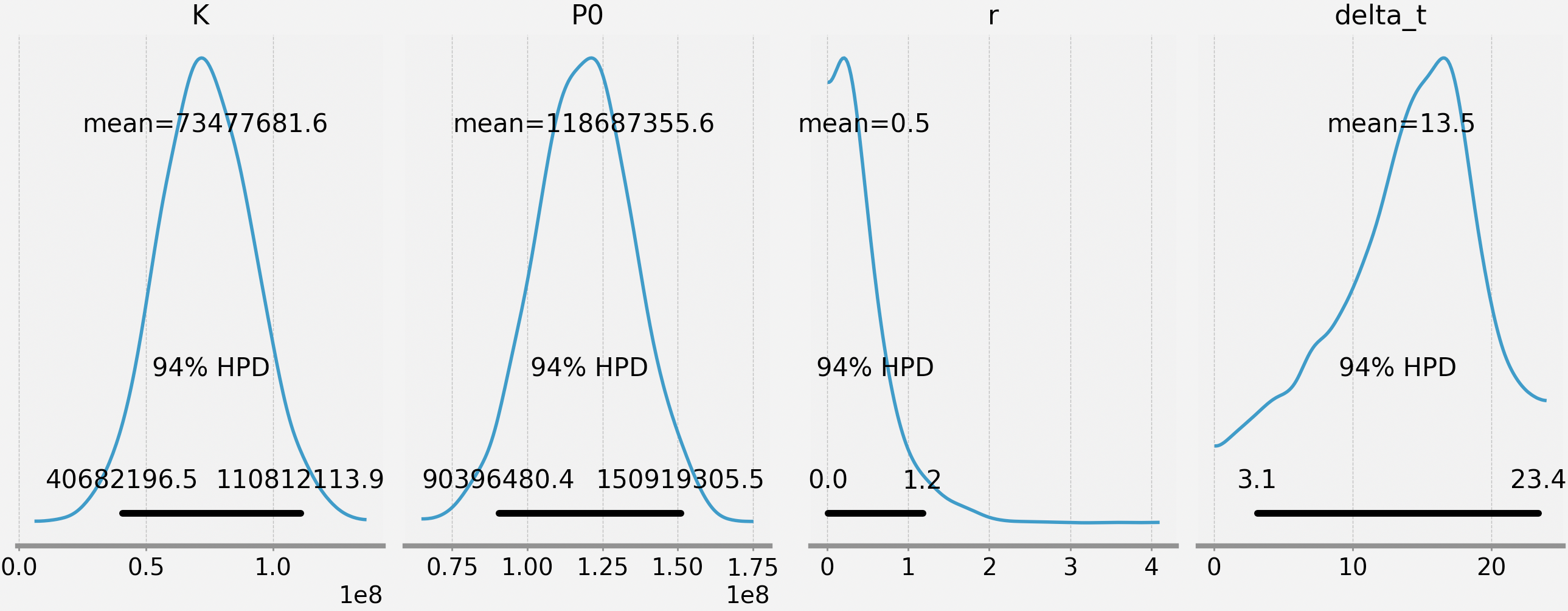Actually, that’s not true: we aren’t really interested in the parameters. Most don’t have an easy interpretation (except for delta_t). What we are really interested in is the posterior of the growth curve. Recall this is a distribution. To demonstrate this, we can sample from the parameters’ posteriors and drop those values into the growth curve. For example, if we sampled 4 times: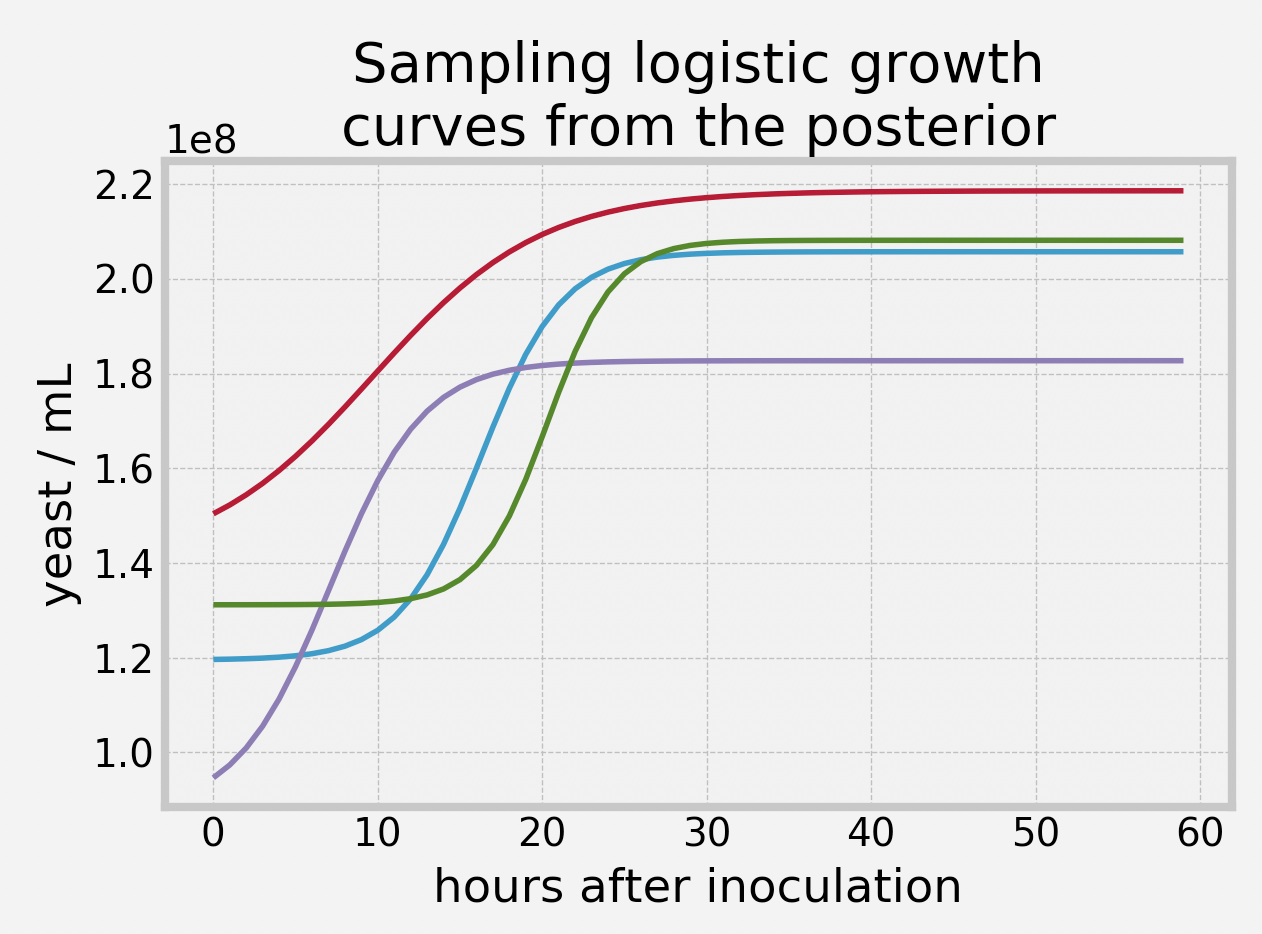Each of these curves look very different, which should give us pause when we make inference about our growth. What happens if we keep sampling growth curves, and then average over all of them - what does that curve look like? What about the error bars on that? This is easy to do (and part of the reason I appreciate Bayesian computation). On the same graph, I’m also going to plot hundreds of potential realizations, as it’s important not to get too focused on the mean as being the “truth” - there is still lots of variation!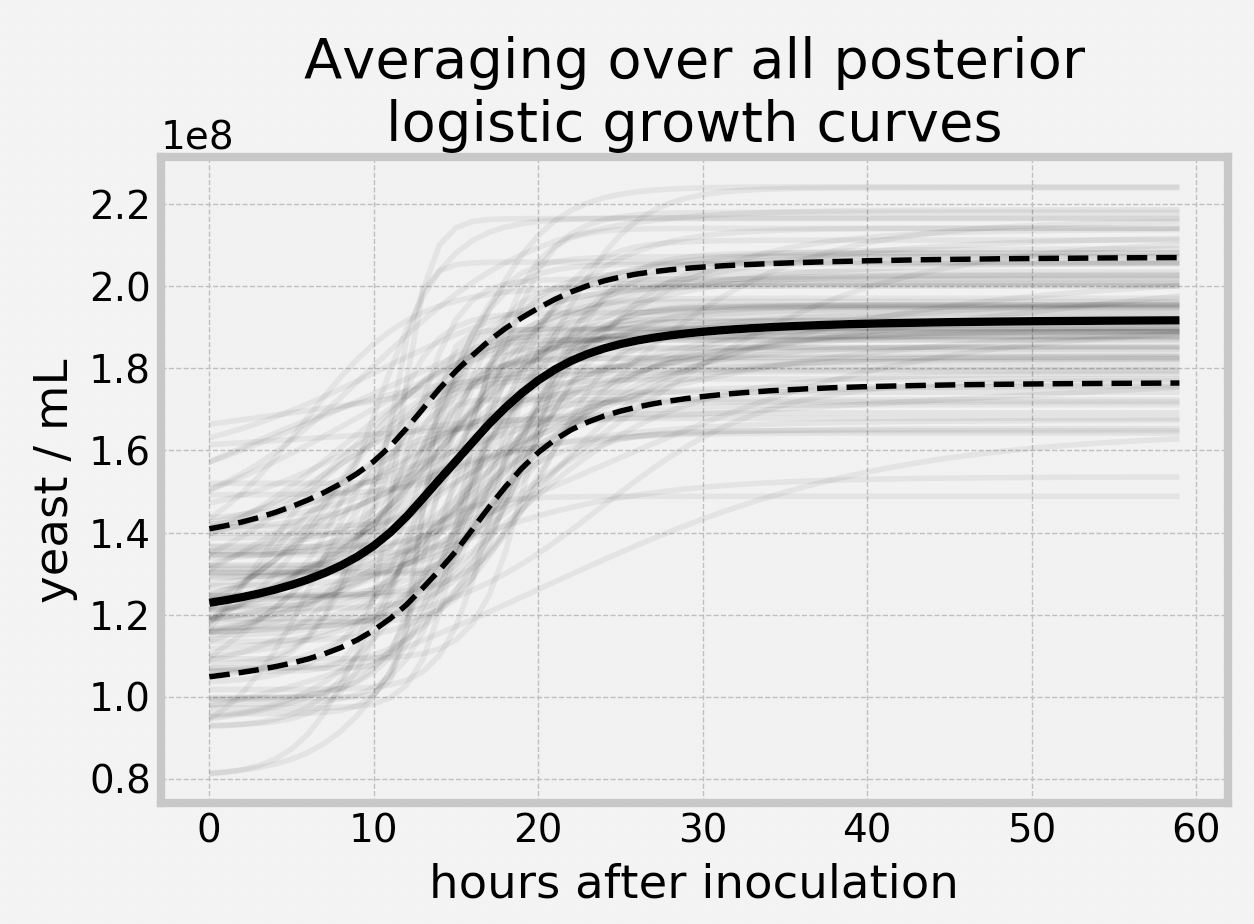Lovely. We can see an estimate for our initial concentration was about 122M ± 18M yeast/mL, and our final concentration was about 191M ± 15M yeast/mL. Eyeballing, the lag phase stopped just prior to 8h (though we need to reconcile this with the posterior mean of the delta_t is 13.5).

What was our estimated yield? I mentioned previously that 50% is expected - how did we do? This computation is also easy in Bayesian inference, we just look at the distribution of the ratio of yeast/mL at time 60 over yeast/mL at time 0. Doing this, the average value of this distribution is 59%.

Conclusion

This was a fun little experiment to mix statistics and biology. Further extensions include adding covariates, and modeling death of cells too (there is a finite amount of energy in the medium, so this should happen eventually). For now, however, I’m washing the yeast and eventually going to dehydrate them.

]]>
https://dataorigami.net/blogs/napkin-folding/bayesian-cell-counting 2019-05-16T18:24:00-04:00 2019-05-23T09:26:01-04:00 Bayesian cell counting Cameron Davidson-Pilon Let’s say you are interested in counting the concentration of cells in some sample. This is a pretty common task: sperm counts, blood cell counts, plankton counts. Microbiologists are always counting. Let’s use the example of yeast counting, which is traditional in beer and wine making. The brewery has a sample of yeast slurry, a highly concentrated amount of yeast, and they would like to know how concentrated it is, so they can add the correct amount to a batch.

The first step is to dilute your sample. The original concentration could be as high as billions per mL, so even a fraction of a mL is going to be still too concentrated to properly count. This is solved by successive dilutions of the original sample:Each time we transfer to a new test tube, we dilute the sample by a factor of 10.

After this is done, a very small amount of the final diluted slurry is added to a hemocytometer. A hecocytometer is a glass slide with a visible grid that an observer can place under a microscope and count cells on. Under the microscope, it looks like this (where yellow dots represent cells):The hemocytometer is designed such that a known quantity of volume exists under the inner 25 squares (usually 0.0001 mL). The observer will apriori pick 5 squares to count cells in, typically the 4 corner squares and the middle square. (Why only 5? Unlike this image above, there could be thousands of cells, and counting all 25 squares would take too long). Since we know the volume of the 25 squares, and the dilution rate, we can recover our original slurry concentration:

$$\text{cells/mL} = (\text{cells counted}) \cdot 5 \cdot (\text{dilution factor}) / 0.0001 \text{mL}$$

Given I counted 49 yeast and my dilution was 1000x, my estimate is 2.45B yeast/mL. Great! We are done, right? No way, consider all the sources of uncertainty we glossed over:

1. Did we accurately measure out exactly 9mL of water in each test tube?
2. Did we accurately extract 1mL of slurry between each test tube?
3. Did we get lucky/unlucky with our 0.0001 mL sample for the hemocytometer?
4. Did the hemocytometer manufacturer have some QA over the volume of the chamber?
5. Did we get lucky/unlucky with cells numbers in the 5 counting squares?

So we should expect high variance in our estimate because of the many sources of noise, and because they are layered on top of one another. Let’s redo this with Bayesian statistics so we can model our uncertainty.

Here’s the code for an observation of 49 yeast cells counted. Each source of noise is a random variable. I’ve added some priors that I think are sensible.

import pymc3 as pm
BILLION = 1e9
TOTAL_SQUARES = 25
squares_counted = 5
yeast_counted = 49
with pm.Model() as model:
yeast_conc = pm.Normal("cells/mL", mu=2 * BILLION, sd=0.4 * BILLION)
shaker1_volume = pm.Normal("shaker1 volume (mL)", mu=9.0, sd=0.05)
shaker2_volume = pm.Normal("shaker2 volume (mL)", mu=9.0, sd=0.05)
shaker3_volume = pm.Normal("shaker3 volume (mL)", mu=9.0, sd=0.05)
yeast_slurry_volume = pm.Normal("initial yeast slurry volume (mL)", mu=1.0, sd=0.01)
shaker1_to_shaker2_volume =    pm.Normal("shaker1 to shaker2 (mL)", mu=1.0, sd=0.01)
shaker2_to_shaker3_volume =    pm.Normal("shaker2 to shaker3 (mL)", mu=1.0, sd=0.01)
dilution_shaker1 = yeast_slurry_volume       / (yeast_slurry_volume + shaker1_volume)
dilution_shaker2 = shaker1_to_shaker2_volume / (shaker1_to_shaker2_volume + shaker2_volume)
dilution_shaker3 = shaker2_to_shaker3_volume / (shaker2_to_shaker3_volume + shaker3_volume)
final_dilution_factor = dilution_shaker1 * dilution_shaker2 * dilution_shaker3
volume_of_chamber = pm.Gamma("volume of chamber (mL)", mu=0.0001, sd=0.0001 / 20)
# why is Poisson justified? in my final shaker, I have yeast_conc * final_dilution_factor * shaker3_volume number of yeast
# I remove volume_of_chamber / shaker3_volume fraction of them, hence it's a binomial with very high count, and very low probability.
yeast_visible = pm.Poisson("cells in visible portion", mu=yeast_conc * final_dilution_factor * volume_of_chamber)
number_of_counted_cells = pm.Binomial("number of counted cells", yeast_visible, squares_counted/TOTAL_SQUARES, observed=yeast_counted)
trace = pm.sample(5000, tune=1000)
pm.plot_posterior(trace, varnames=['cells/mL'])

The posterior for cells/mL is below: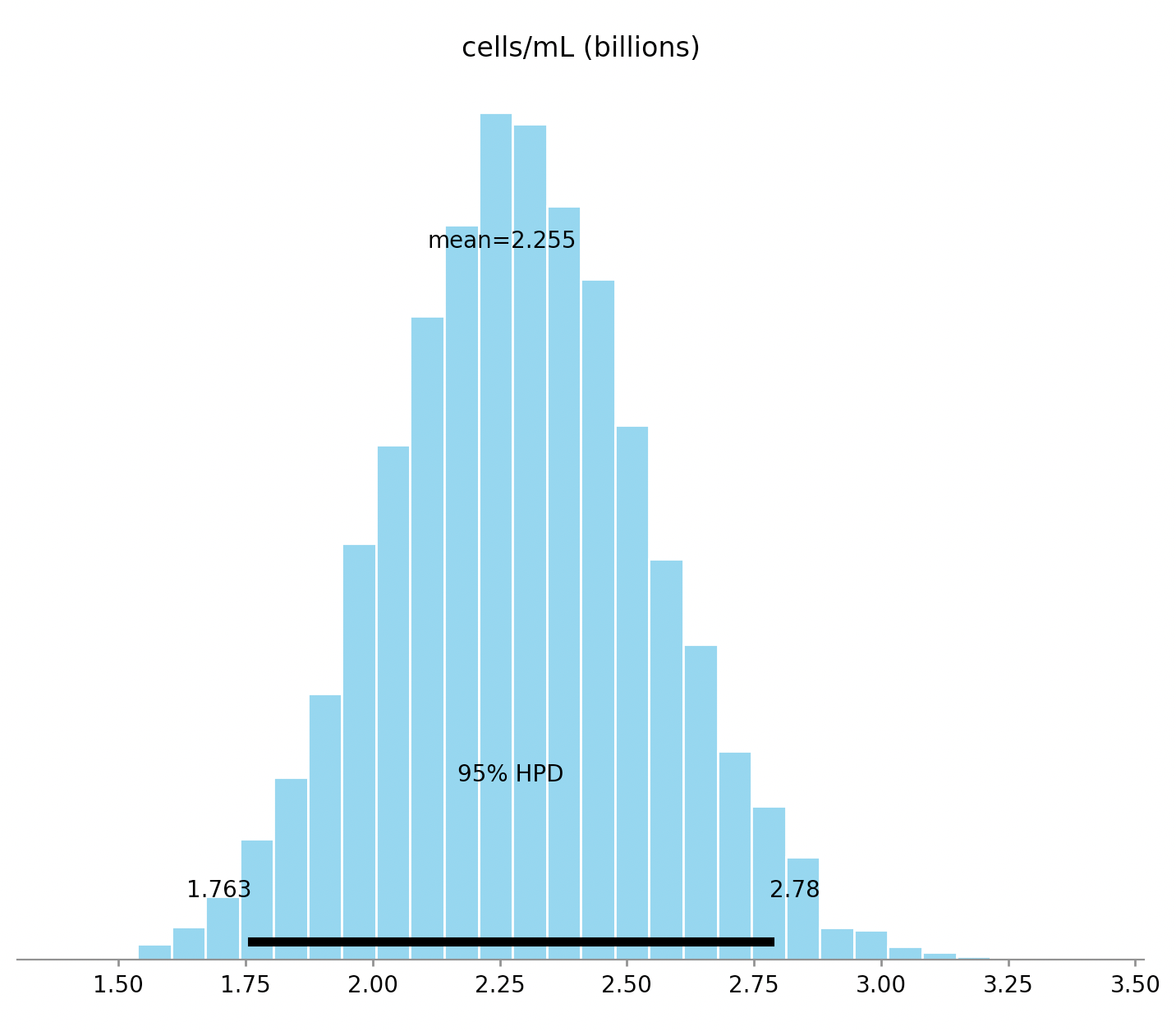We can see that the width of the credible interval is about a billion. That’s surprisingly large. And that makes sense: we only have a single, noisy observation. We can see the influence of the prior here as well. Note that the posterior’s mean is about 2.25B, much closer to the priors’ mean of 2B than our naive estimate of 2.45B above. This brings up another point: using the naive formula above is like saying: “I observed a single coin flip, saw heads, so all coin flips are heads.” Sounds silly, but it’s identical inference. With Bayesian statistics, we get to lie in a much more reassuring bed!

Read the next article in this series, modelling growth

]]>
https://dataorigami.net/blogs/napkin-folding/churn 2019-04-27T11:08:00-04:00 2020-08-03T16:00:42-04:00 SaaS churn and piecewise regression survival models Cameron Davidson-Pilon A software-as-a-service company (SaaS) has a typical customer churn pattern. During periods of no billing, the churn is relatively low compared to periods of billing (typically every 30 or 365 days). This results in a distinct survival function for customers. See below:

kmf = KaplanMeierFitter().fit(df['T'], df['E'])
kmf.plot(figsize=(11,6));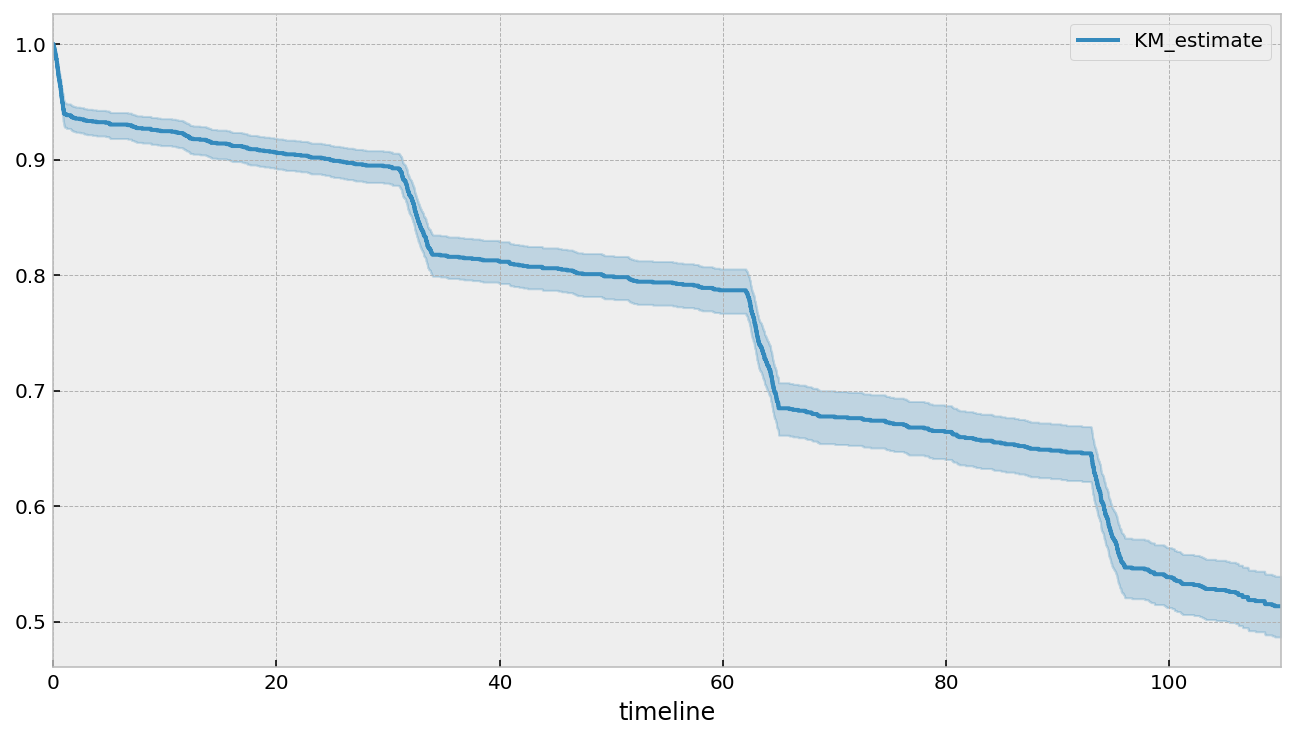To borrow a term from finance, we clearly have different regimes that a customer goes through: periods of low churn and periods of high churn, both of which are predictable. This predictability and "sharp" changes in hazards suggests that a piecewise hazard model may work well: hazard is constant during intervals, but varies over different intervals.

Furthermore, we can imagine that individual customer variables influence their likelihood to churn as well. Since we have baseline information, we can fit a regression model. For simplicity, let's assume that a customer's hazard is constant in each period, however it varies over each customer (heterogeneity in customers). Hat tip to StatWonk for this model:

Our hazard model looks like¹: $$h(t\;|\;x) = \begin{cases} \lambda_0(x)^{-1}, & t \le \tau_0 \\ \lambda_1(x)^{-1} & \tau_0 < t \le \tau_1 \\ \lambda_2(x)^{-1} & \tau_1 < t \le \tau_2 \\ ... \end{cases}$$

and $$\lambda_i(x) = \exp(\mathbf{\beta}_i x^T), \;\; \mathbf{\beta}_i = (\beta_{i,1}, \beta_{i,2}, ...)$$. That is, each period has a hazard rate, $$\lambda_i$$, that is the exponential of a linear model. The parameters of each linear model are unique to that period - different periods have different parameters (later we will generalize this).

Why do I want a model like this? Well, it offers lots of flexibility (at the cost of efficiency though), but importantly I can see:

1. Influence of variables over time.
2. Looking at important variables at specific "drops" (or regime changes). For example, what variables cause the large drop at the start? What variables prevent death at the second billing?
3. Predictive power: since we model the hazard more accurately (we hope) than a simpler parametric form, we have better estimates of a subjects survival curve.

One interesting point is that this model is not an accelerated failure time model even though the behaviour in each interval looks like one. This is because the breakpoints (intervals) do not change in response (contract or dilate) to the covariates (but that's an interesting extension).

¹ I specify the reciprocal because that follows lifelines convention for exponential and Weibull hazards. In practice, it means the interpretation of the sign is possibly different.

pew = PiecewiseExponentialRegressionFitter(
breakpoints=breakpoints)\
.fit(df, "T", "E")

Above we fit the regression model. We supplied a list of breakpoints that we inferred from the survival function and from our domain knowledge.

Let's first look at the average hazard in each interval, over time. We should see that during periods of high customer churn, we also have a high hazard. We should also see that the hazard is constant in each interval.

fig, ax = plt.subplots(1,1)
kmf.plot(figsize=(11,6), ax=ax);
ax.legend(loc="upper left")
ax.set_ylabel("Survival")
ax2 = ax.twinx()
pew.predict_cumulative_hazard(
pew._norm_mean.to_frame(name='average hazard').T,
times=np.arange(0, 110),
).diff().plot(ax=ax2, c='k', alpha=0.80)
ax2.legend(loc="upper right")
ax2.set_ylabel("Hazard")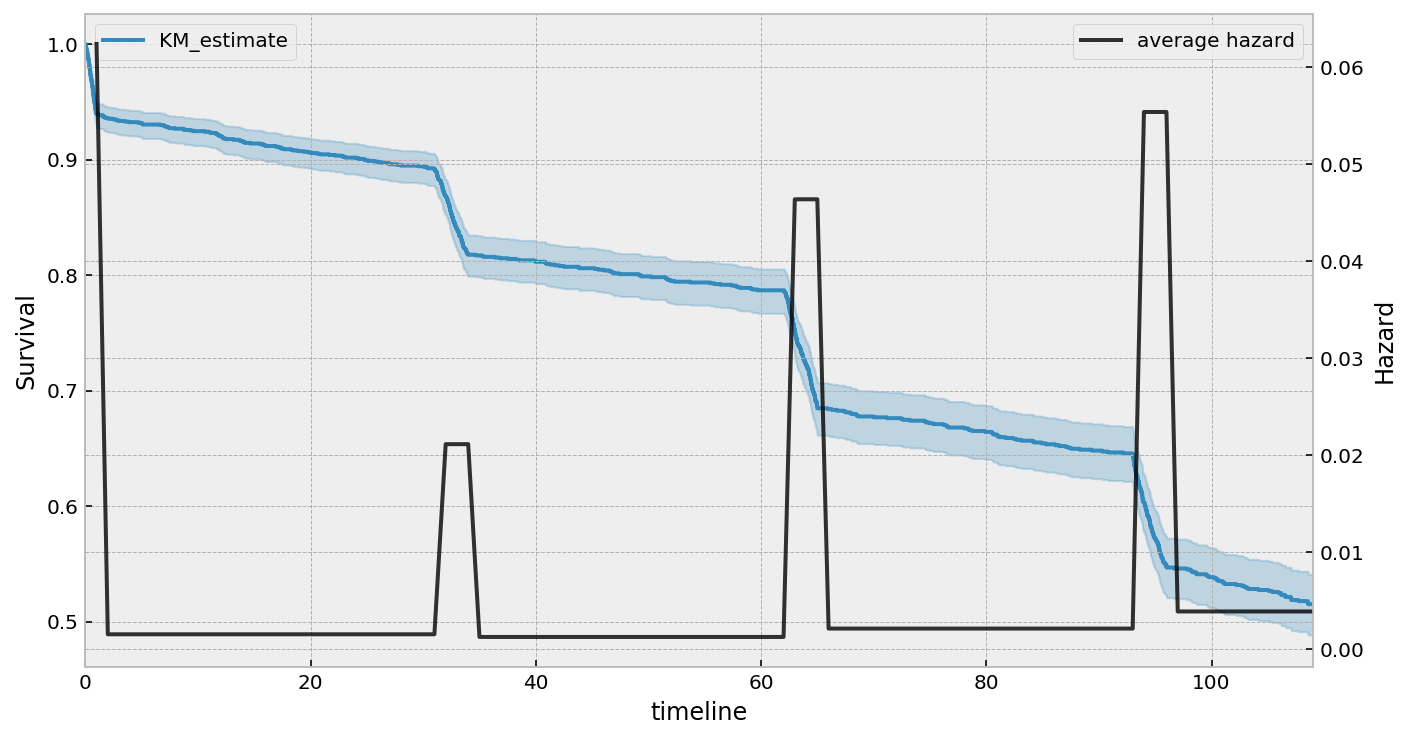It's obvious that the highest average churn is in the first few days, and then high again in the latter billing periods.

So far, we have only been looking at the aggregated population - that is, we haven't looked at what variables are associated with churning. Let's first start with investigating what is causing (or associated with) the drop at the second billing event (~day 30).

fig, ax = plt.subplots(figsize=(10, 4))
pew.plot(parameter=['lambda_2_'], ax=ax);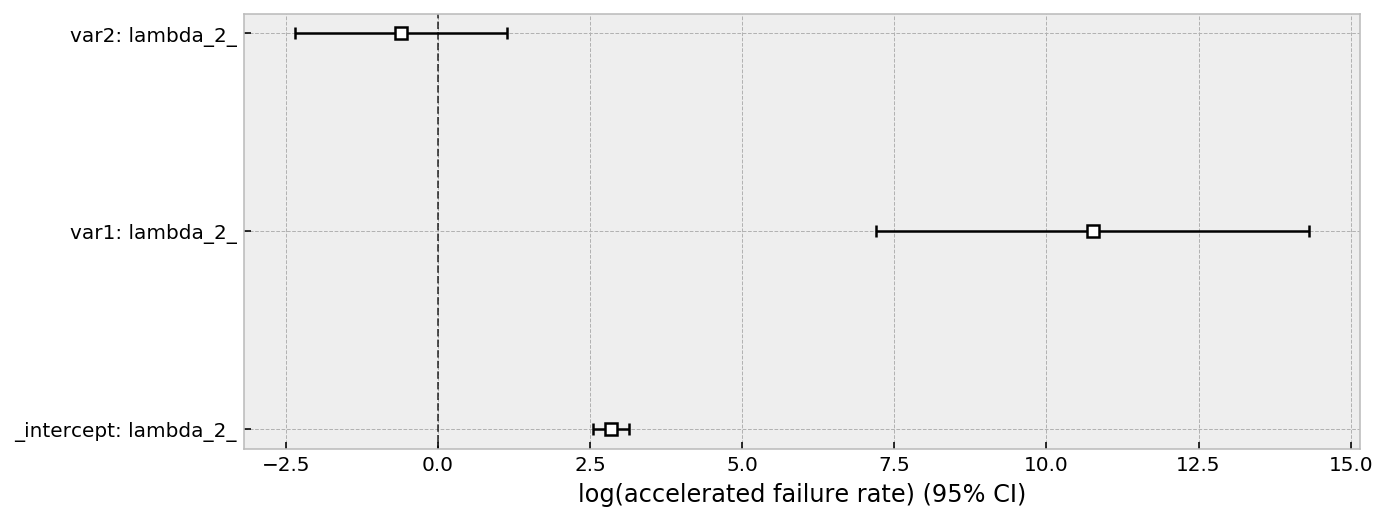From this forest plot, we can see that the var1 has a protective effect, that is, customers with a high var1 are much less likely to churn in the second billing periods. var2 has little effect, but possibly negative. From a business point of view, maximizing var1 for customers would be a good move (assuming it's a causal relationship).

We can look at all the coefficients in one large forest plot, see below. We see a distinct alternating pattern in the _intercepts variable. This makes sense, as our hazard rate shifts between high and low churn regimes. The influence of var1 seems to spike in the 3rd interval (lambda_2_), and then decays back to zero. The influence of var2 looks like it starts to become more negative over time, that is, is associated with more churn over time.

fig, ax = plt.subplots(figsize=(10, 10))
pew.plot(ax=ax);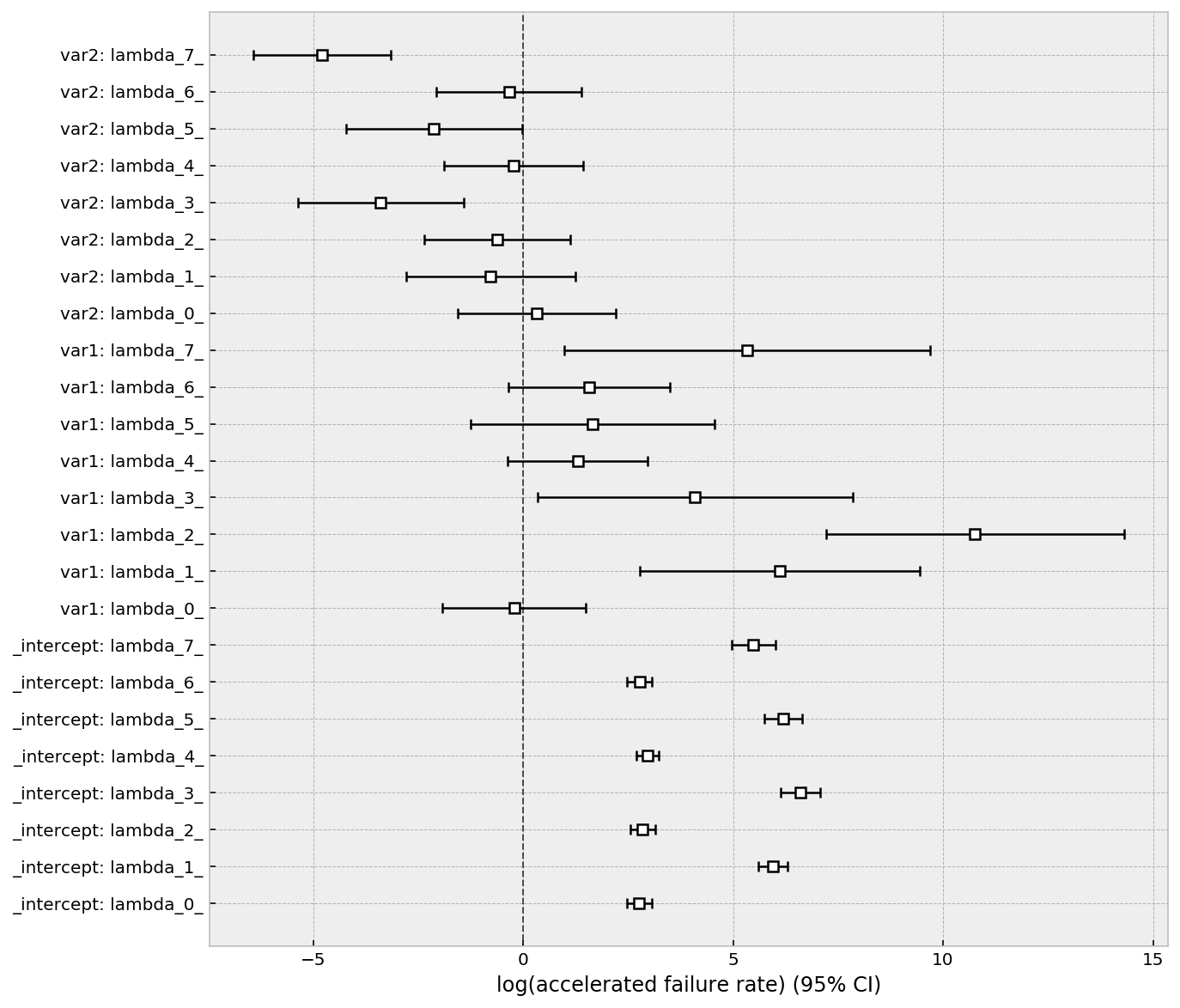Regularization as a model parameter

If we suspect there is some parameter sharing between intervals, or we want to regularize (and hence share information) between intervals, we can include a penalizer which penalizes the variance of the estimates per covariate.

Note: we do not penalize the intercept, currently. This is a modelers decision, but I think it's better not too.

Specifically, our penalized log-likelihood, $$PLL$$, looks like:

$$PLL = LL - \alpha \sum_j \hat{\sigma}_j^2$$

where $$\hat{\sigma}_j$$ is the standard deviation of $$\beta_{i, j}$$ over all periods $$i$$. This acts as a regularizer and much like a multilevel component in Bayesian statistics. In the above inference, we implicitly set $$\alpha$$ equal to 0. Below we examine some more cases of varying $$\alpha$$. First we set $$\alpha$$ to an extremely large value, which should push the variances of the estimates to zero.

# Extreme case, note that all the covariates' parameters are almost identical.
pew = PiecewiseExponentialRegressionFitter(
breakpoints=breakpoints,
penalizer=20.0)\
.fit(df, "T", "E")
fig, ax = plt.subplots(figsize=(10, 10))
pew.plot(ax=ax);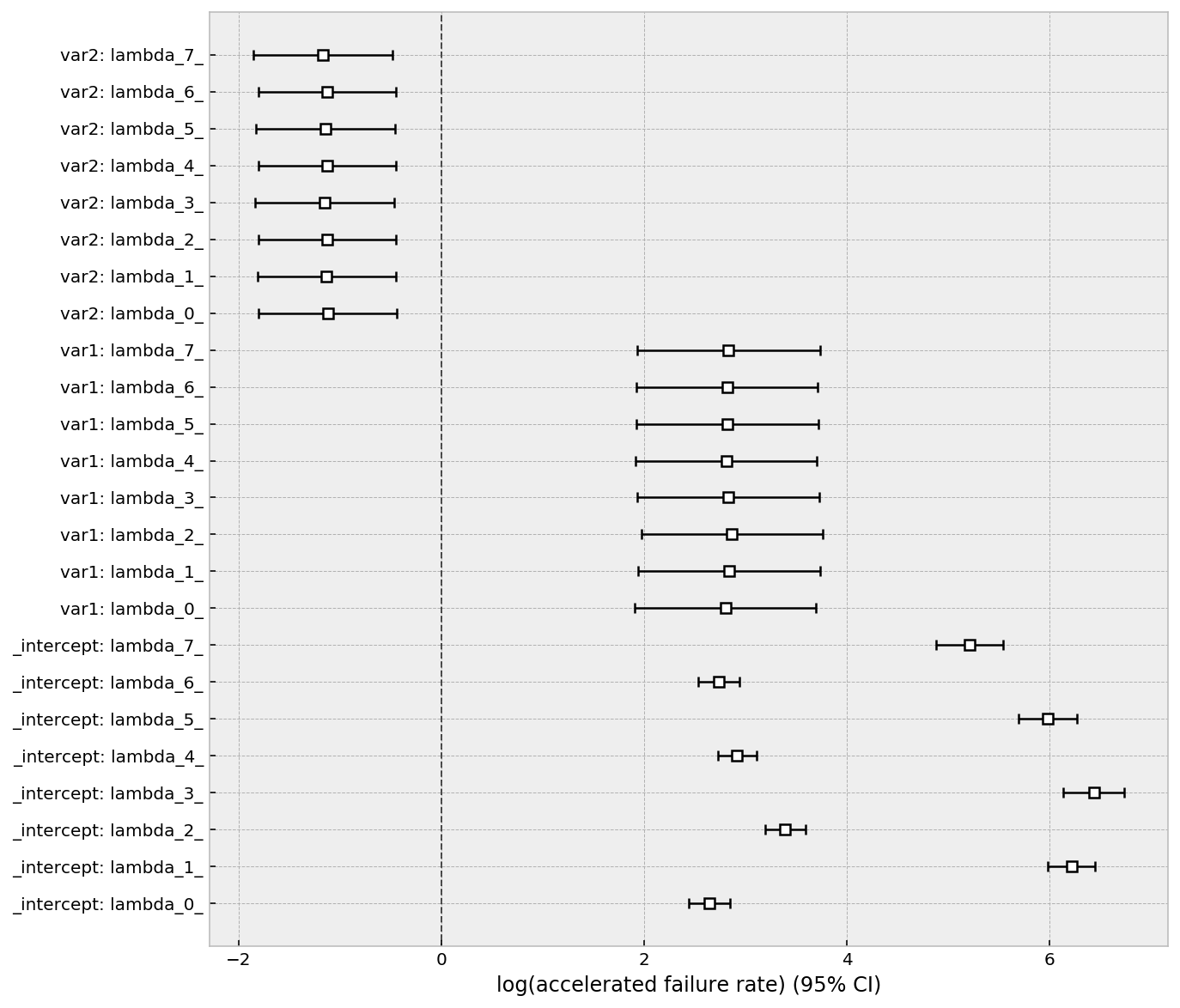As we suspected, a very high penalizer will constrain the same parameter between intervals to be equal (and hence 0 variance). This is the same as the model:

$$h(t\;|\;x) = \begin{cases} \lambda_0(x)^{-1}, & t \le \tau_0 \\ \lambda_1(x)^{-1} & \tau_0 < t \le \tau_1 \\ \lambda_2(x)^{-1} & \tau_1 < t \le \tau_2 \\ ... \end{cases}$$

and $$\lambda_i(x) = \exp(\mathbf{\beta_{0,i} + \beta} x^T), \;\; \mathbf{\beta} = (\beta_{1}, \beta_{2}, ...)$$. Note the reuse of the $$\beta$$s between intervals.

This model is the same model proposed in "Piecewise Exponential Models for Survival Data with Covariates".

One nice property of this model is that because of the extreme information sharing between intervals, we have maximum information for inferences, and hence small standard errors per parameter. However, if the parameters effect is truly time-varying (and not constant), then the standard error will be inflated and a less constrained model is better.

Below we examine a in-between penalty, and compare it to the zero penalty.

# less extreme case
pew = PiecewiseExponentialRegressionFitter(
breakpoints=breakpoints,
penalizer=.25)\
.fit(df, "T", "E")
fig, ax = plt.subplots(figsize=(10, 10))
pew.plot(ax=ax, fmt="s", label="small penalty on variance")
# compare this to the no penalizer case
pew_no_penalty = PiecewiseExponentialRegressionFitter(
breakpoints=breakpoints,
penalizer=0)\
.fit(df, "T", "E")
pew_no_penalty.plot(ax=ax, c="r", fmt="o", label="no penalty on variance")
plt.legend();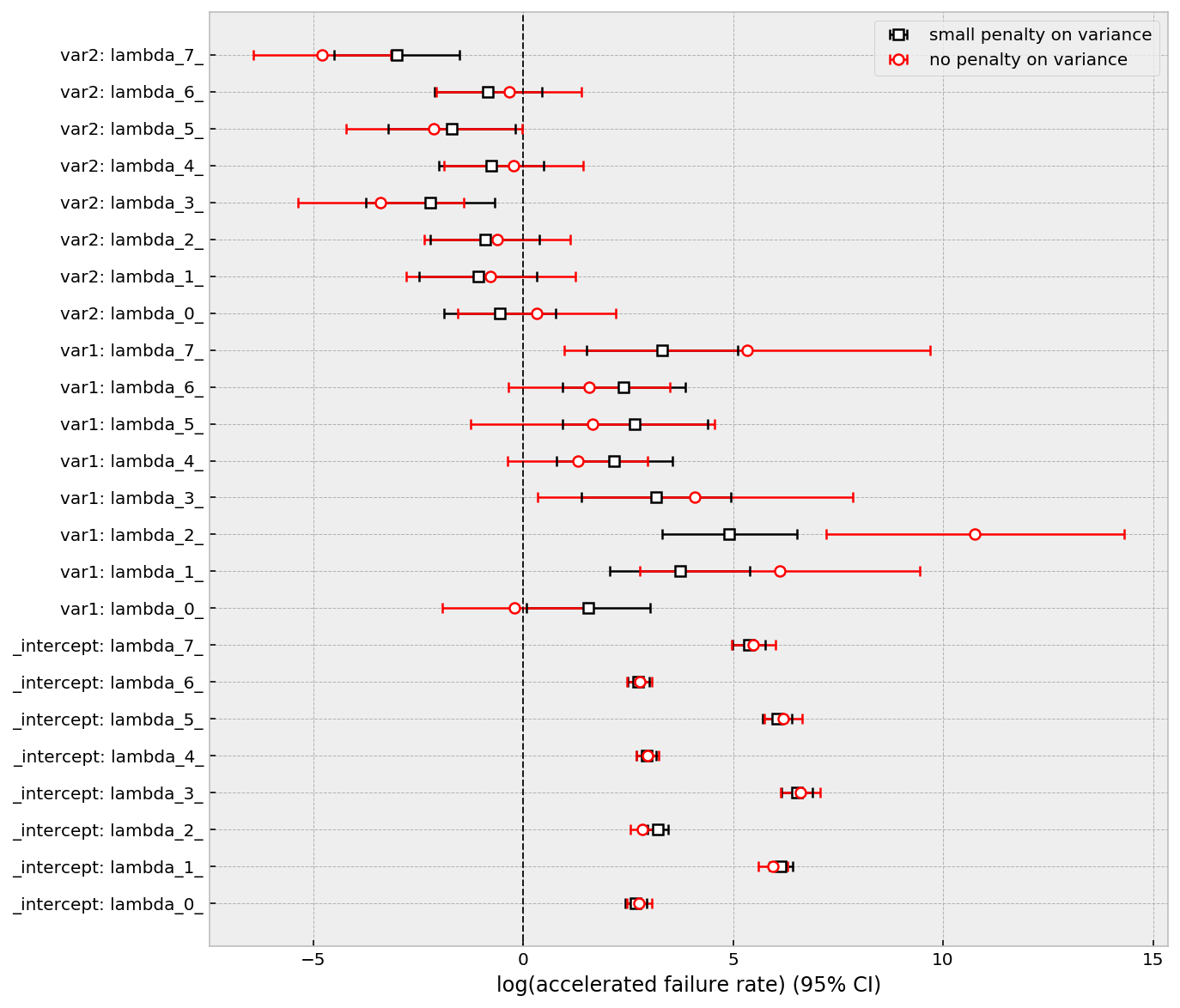We can see that:

1. on average, the standard errors are smaller in the penalty case
2. parameters are pushed closer together (they will converge to their average if we keep increasing the penalty)
3. the intercepts are barely effected.

I think, in practice, adding a small penalty is the right thing to do. It's extremely unlikely that intervals are independent, and extremely unlikely that parameters are constant over intervals.

Like all lifelines models, we have prediction methods too. This is where we can see customer heterogeneity vividly.

# Some prediction methods
pew.predict_survival_function(df.loc[0:3]).plot(figsize=(10, 5));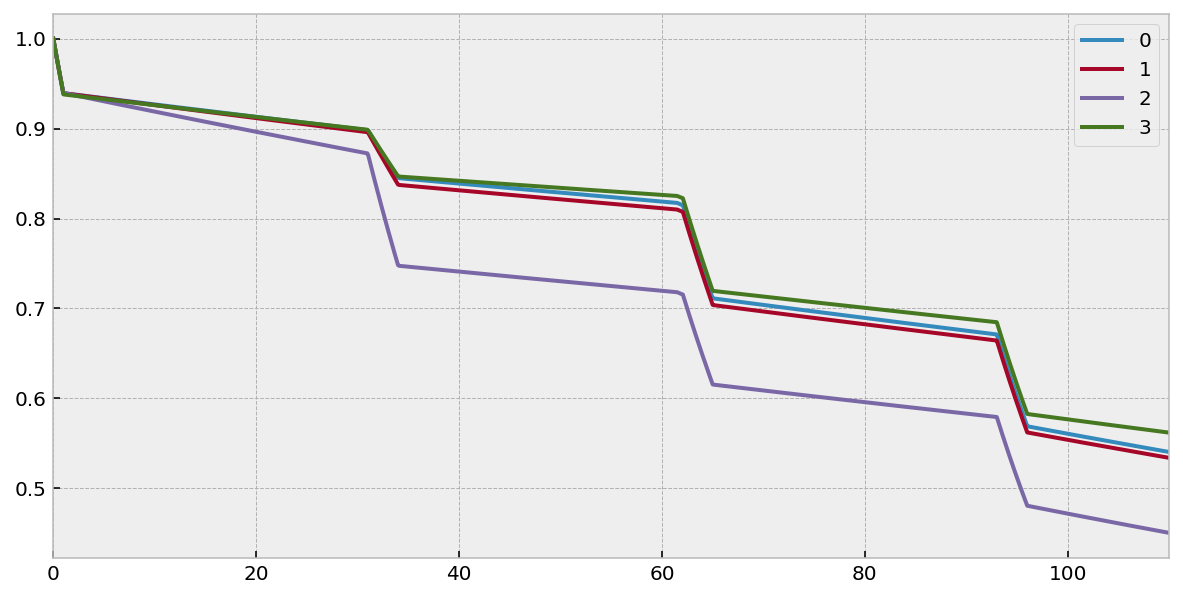pew.predict_cumulative_hazard(df.loc[0:3]).plot(figsize=(10, 5));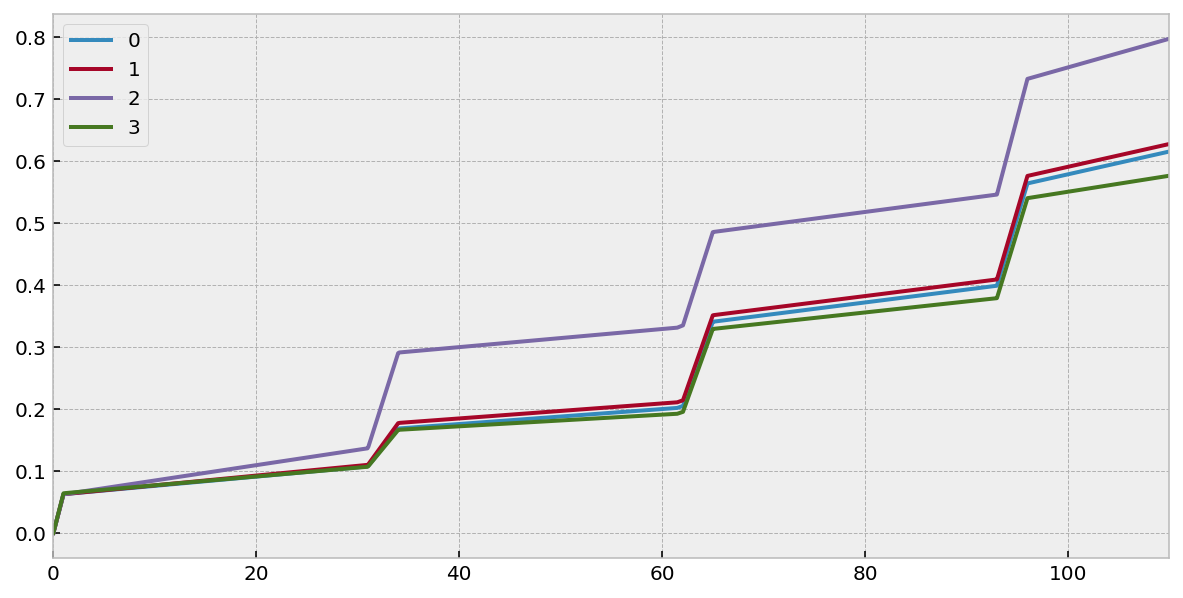pew.predict_median(df.loc[0:5])
0.5
0 inf
1 inf
2 95.380584
3 inf
4 inf
5 inf

Conclusion

In conclusion, this model is pretty flexible and it is one that can encourage more questions to be asked. Beyond just SaaS churn, one can think of other application of piecewise regression models: employee churn after their stock option vesting cliff, mortality during different life stages, or modelling time-varying parameters.

Future extensions include adding support for time-varying covariates. Stay tuned!

]]>
https://dataorigami.net/blogs/napkin-folding/counting-and-interval-censoring 2019-04-09T17:05:00-04:00 2019-08-06T08:47:28-04:00 Counting and interval censoring analysis Cameron Davidson-Pilon Let’s say you have an initial population of (micro-)organisms, and you are curious about their survival rates. A common summary statistic of their survival is the half-life. How might you collect data to measure their survival? Since we are dealing with micro-organisms, we can’t track individual lifetimes. What we might do is periodically count the number of organisms still alive. Suppose our dataset looks like:

T = [0,    2,   4,   7  ]  # in hours
N = [1000, 914, 568, 112]

I’ll present two so-so solutions to finding the half-life, and then one much better solution.

Solution 1: Linear interpolation

We can plot this over time: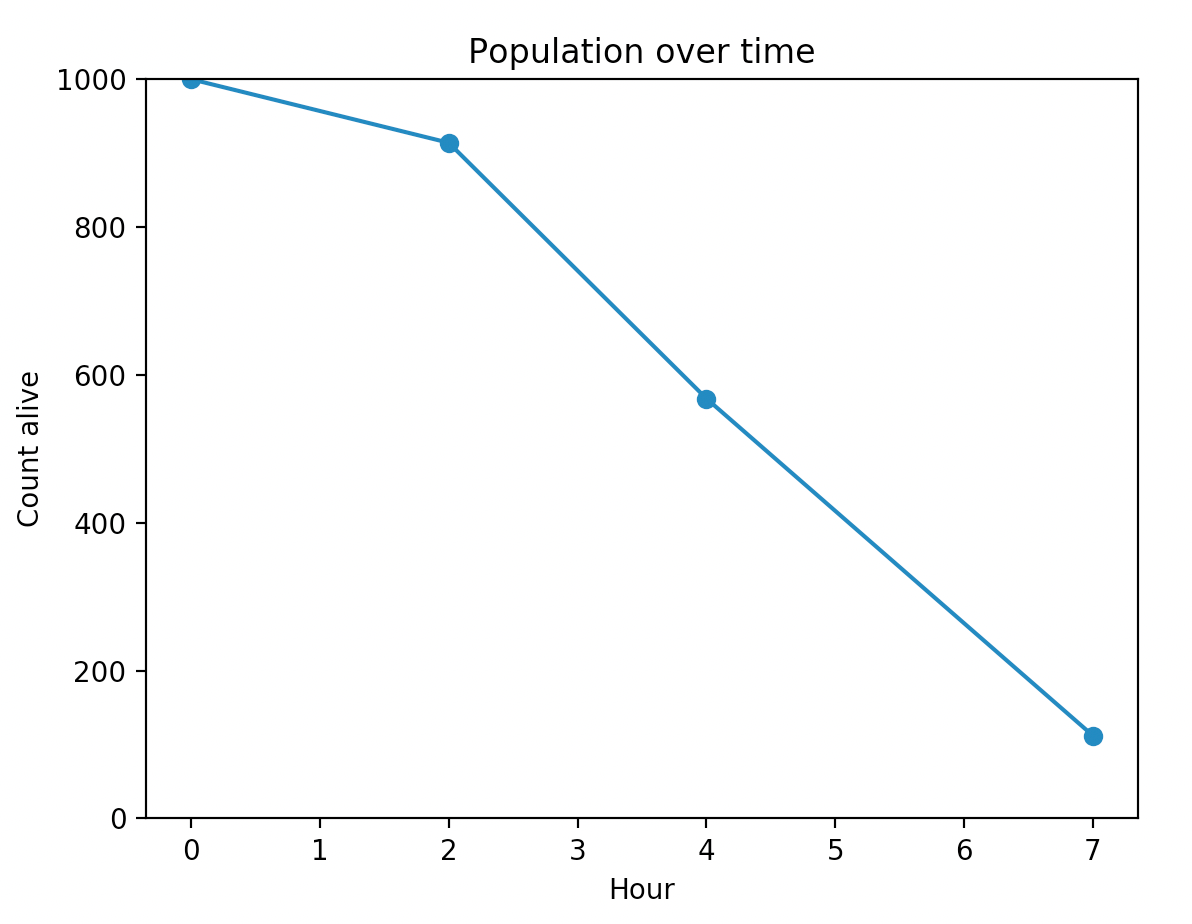We can eyeball the half-life to be about 4.3h - note that this is a linear interpretation between two points, and thus this method doesn’t consider all the data (i.e. it’s not a global method).

Solution 2: curve-fitting an exponential model

Exponential death is a common model in ecology because of it’s simplicity. We can try to find a $$\beta$$ value such that the sum of squares of observed values minus $$1000 \exp(\beta t)$$ is minimized. That’s not hard to do with scipy’s minimize functions. The best estimate for $$\beta$$ is about 0.17. But, as we can see, it’s not a very good fit:We could choose a more flexible model (like a Weibull distribution), but we will still run up against a fundamental problem: the variance on the estimates will be very high because we are only considering 4 data points, and adding more parameters to the fitting model will only make things worse.

Solution 3: consider the entire population and censoring

Think again about how the data was collected for a moment. We took a count, waited a few hours, and then took a count again. The delta in the population are composed of individuals that died sometime in that interval. So what we have is a case of interval censoring. That is, I know that 86 individuals died sometime between 0 and 2 (lower and upper bound), 346 died sometime between 2 and 4 (lower and upper bound), etc. Finally, we are left with 112 that are right censored, that is, we stopped watching and don’t observe their death.

We can use survival analysis to answer this problem (hey, let’s use lifelines!). Importantly, we can treat all 1000 organisms as individual observations to make the inference stronger. First, we’ll do some data manipulation.

df = pd.DataFrame({
"deltas": [86, 346, 456, 112],
"start":  [0,  2,   4,   7],
"stop":   [2,  4 ,  7,   np.inf],
"observed_death": [False, False, False, False]
})
print(df)
"""
deltas  start  stop  observed_death
0      86      0   2.0           False
1     346      2   4.0           False
2     456      4   7.0           False
3     112      7   inf           False
"""

We have observed_death as always False, because we don’t have exact measurements on any of the deaths (that is, I have no organisms where I can say “this guy lived exactly X hours”). Why do I have the infinity in the last row? Well, we don’t observe the final 112 organisms die either, but we know they will die between hour 7 and infinity, so we code that in too.

from lifelines import WeibullFitter
wf = WeibullFitter()
wf.fit_interval_censoring(
df['start'],
df['stop'],
df['observed_death'],
weights=df['deltas']
)
wf.print_summary()
"""
<lifelines.WeibullFitter: fitted with 4 observations, 4 censored>
number of subjects = 4
number of events = 0
log-likelihood = -1182.357
hypothesis = lambda_ != 1, rho_ != 1
---
coef  se(coef)  lower 0.95  upper 0.95      p  -log2(p)
lambda_  5.09      0.07        4.94        5.23 <0.005       inf
rho_     2.49      0.08        2.35        2.64 <0.005    282.95
"""

Note the very small standard errors. And if we plot the resulting survival curve, we see an excellent fit.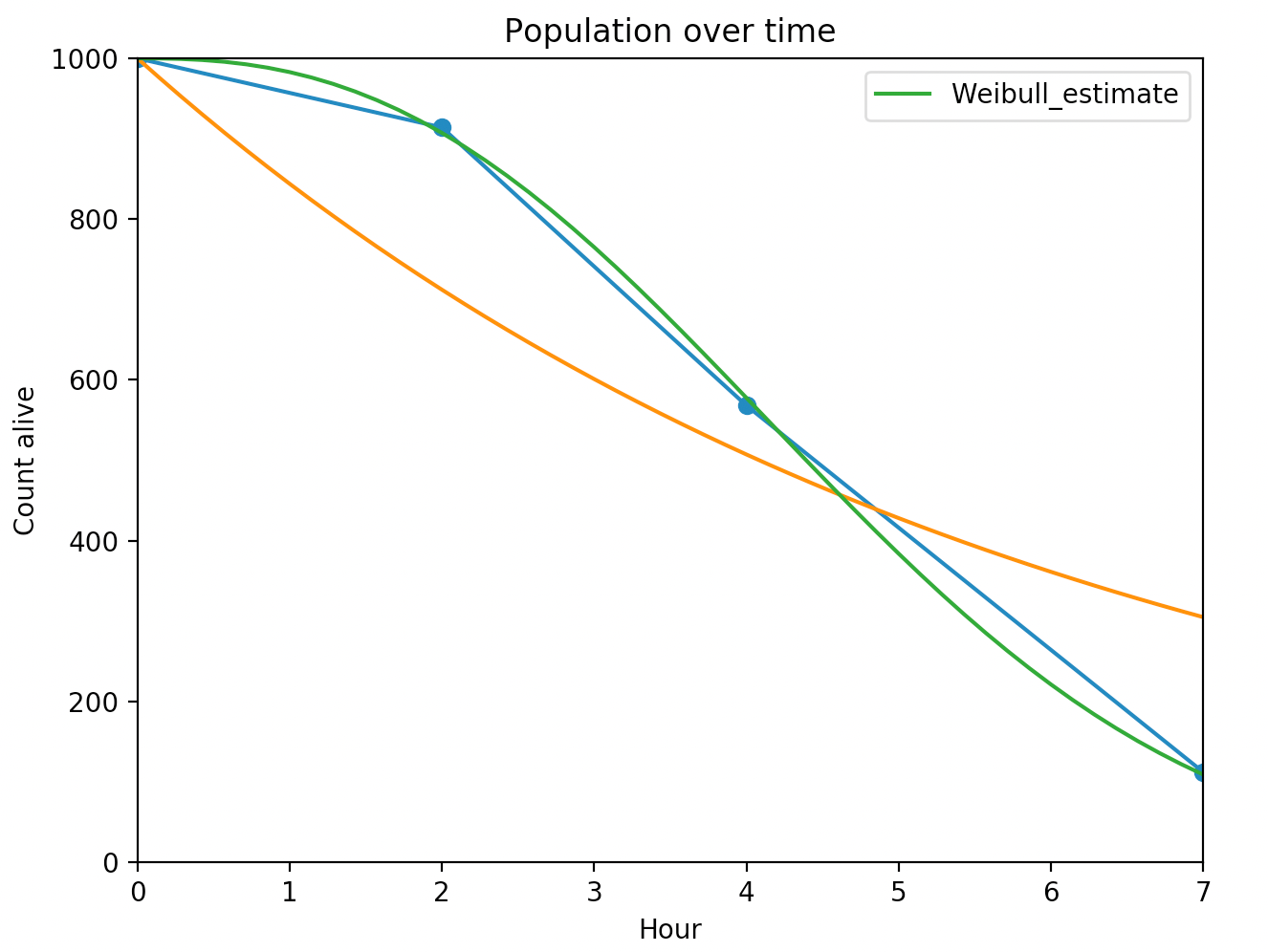From this, we can report the half-life, or even better is to present the survival distribution, since it has the most information in it.

Multiple cultures

Let's say we actually have two cultures we are working with, and take population measurements at the same time. However we have different environments for each of the cultures: one has double the sugar concentration in the medium than the other. We can model this using a regression survival model:

culture_1 = pd.DataFrame({
"deltas": [86, 346, 456, 112],
"start":  [0,  2,   4,   7],
"stop":   [2,  4 ,  7,   np.inf],
"conc_sugars": [0.15, 0.15, 0.15, 0.15],
"observed_death": [False, False, False, False]
})
culture_2 = pd.DataFrame({
"deltas": [50, 202, 566, 182],
"start":  [0,  2,   4,   7],
"stop":   [2,  4 ,  7,   np.inf],
"conc_sugars": [0.30, 0.30, 0.30, 0.30],
"observed_death": [False, False, False, False]
})
df = pd.concat([culture_2, culture_1])
from lifelines import WeibullAFTFitter
w_aft = WeibullAFTFitter().fit_interval_censoring(df, lower_bound_col='start',
upper_bound_col='stop',
event_col='observed_death',
weights_col='deltas')

w_aft.params_

"""
lambda_ conc_sugars 0.901763
_intercept 1.499147
rho_ _intercept 0.996401

"""

So what is the impact of sugar on microorganisms death? The coefficient associated with sugar concentration in this AFT model is interpreted as "higher means live longer". We get a coefficient of 0.90. Thus organisms with 0.15 sugar concentration "experience" time at a 14.4% faster rate than organisms with 0.30 sugar concentration (Why? $$\exp(0.90 (0.30-0.15))=1.144$$ ).

Conclusion

This is a simple case of when you want to use interval censoring instead of more naive ways. By thinking about the data generating process, we gain lots of statistical efficiency and better predictions.

]]>
https://dataorigami.net/blogs/napkin-folding/the-delta-method-and-autograd 2019-03-27T11:49:00-04:00 2019-05-22T22:41:54-04:00 The Delta-Method and Autograd Cameron Davidson-Pilon One of the reasons I’m really excited about autograd is because it enables me to be able to transform my abstract parameters into business-logic. Let me explain with an example. Suppose I am modeling customer churn, and I have fitted a Weibull survival model using maximum likelihood estimation. I have two parameter estimates: lambda-hat and rho-hat. I also have their covariance matrix, which tells me how much uncertainty is present in the estimates (in lifelines, this is under the variance_matrix_ property). From this, I can plot the survival curve, which is fine, but what I really want is a measure of lifetime value.

Suppose customers give us $10 each time period for the first 3 time periods, and then$30 each time period afterwards. For a single user, the average LTV (up to timeline) calculation might look like:

# create a Weibull model with fake data
wf = WeibullFitter().fit(np.arange(1, 100))
from autograd import numpy as np
def LTV(params, timeline):
lambda_, rho_ = params
sf = np.exp(-(timeline/lambda_) ** rho_)
clv = 10 * (timeline <= 3) * sf + 30 * (timeline > 3) * sf
return clv.sum()
timeline = np.arange(10)
params = np.array([wf.lambda_, wf.rho_])
LTV(params, timeline)
# 214.76

This gives me a point estimate. But I also want the variance, since there is uncertainty in lambda-hat and rho-hat. There is a technique called the delta-method that allows me to transform variance from one domain to another. But to use that, I need the gradient of LTV w.r.t to lambda and rho. For this problem, I could probably write out the gradient, but it would be messy and error-prone. I can use autograd instead to do this.

# array([ 0.15527043, 10.78900217])

The output of the above is the gradient at the params value. Very easy. Now, if I want the variance of the LTV, the delta methods tells me to multiply gradient by the covariance matrix and the gradient’s transpose (check: this should return a scalar and not a vector/matrix):

.dot(wf.variance_matrix_)\

So, my best estimate of LTV (at timeline=10) is 214.76 (3.127). From this, we can build confidence intervals, etc.

]]>
https://dataorigami.net/blogs/napkin-folding/evolution-of-lifelines-over-the-past-few-months 2019-03-13T16:20:00-04:00 2019-10-23T10:52:26-04:00 Evolution of lifelines over the past few months Cameron Davidson-Pilon

TLDR: upgrade lifelines for lots of improvements

pip install -U lifelines

During my time off, I’ve spent a lot of time improving my side projects so I’m at least kinda proud of them. I think lifelines, my survival analysis library, is in that spot. I’m actually kinda proud of it now.

A lot has changed in lifelines in the past few months, and in this post I want to mention some of the biggest additions and the stories behind them.

Performance improvements to Cox model

The Cox proportional hazard model is the workhorse of survival analysis. Almost all papers, unless good reason not to, use the Cox model. This was one of the first regression models added to lifelines, but it has always been too slow. It’s implemented in Numpy, but there was a tricky for loop still in Python. I had ideas on how to turn that loop into a vectorized Numpy operation of matrix products, but there would have been an intermediate variable that created a d x d x p tensor, where d is the number of independent covariates and p is the size of some subset of subjects (at worst, p could be equal to the number of subjects). This will quickly explode the amount of memory required and hence performance would degrade.

One night, on Twitter, I noticed some posts about how to use einsum in PyTorch and Numpy. I had previously heard of it, but didn’t think it was something that was implemented in Numpy nor did I think it was something I could use. Turns out, einsum is a way to do matrix multiplication without intermediate variables! How? Since a product of matrices is a just multiplications and sums, one can declaratively define what the end product should look like and the internals of einsum will compute the intermediate operations at the C layer (I’m simplifying and my own understanding of this is shaky). Suffice to say, after racking my brain and lots of trial and error with einsum, I could replace the tricky Python for loop with einsum! This resulted in a 3x performance increase. However, what I've gained in performance, I've lost in some readability of my code. I’ve put some references to einsum in the bottom of this article.

The second significant improvement to the performance of the Cox model is using a meta-algorithm to select the fastest algorithm. There are two ways to compute the likelihood, and the performance of each is highly dependent on a few characteristics of the dataset, notably how many ties are in the dataset. After running some tests, I noticed that the delineation of when one was faster than the other was not clear, so a heuristic like if num_ties > 10: would not be very effective. What I did instead was to generate hundreds of artificial dataset of varying ties and varying size, and measured the timing of both algorithms. I then fit a linear model to the ratio of the times, conditioned on the ties and size (and their interaction). It was a surprisingly good fit! So now in lifelines, at runtime, I compute some statistics about the incoming dataset, plug these values into a fitted linear model, and the result is the predicted ratio of timings between the two algorithms. I then choose which algorithm would be faster and continue on. The prediction is super fast (it’s a linear model after all), so there are no performance hits there. With this meta-algorithm, the lifelines Cox implementation is up to 3x faster for some datasets. I wrote up a full summary of the idea in a previous blog post .

Overall, the Cox model is now 10x faster than it was a few months ago. (Also, I only mention it here, but the Aalen Additive model is like 50x times faster, but most of those speed improvements where replacing Pandas with NumPy in critical points.)

One large gap in lifelines was checking the proportional hazards assumption, which is critical for any kind of inference-focused modeling (it matters less for prediction tasks). The author of the popular R survival library, Terry Therneau, has made massive contributions to survival analysis techniques, including a statistical test for non-proportionality. This test relies on an important residual of the Cox regression. While implementing this statistical test in lifelines, I realized there was a more general solution for handle all residuals, so I added functionality to compute the most common residuals.

These additions enabled a new, very user friendly function, check_assumptions, which prints out potential proportionality violations in human readable format and offers advice on how to fix it. I also introduced residuals plots:I think too many people are focused on deep learning. There, I said it, and probably you agree. However, some really cool technologies are falling out of that area that others can use. One of them is libraries that implement automatic differentiation, aka autodiff. This is like the holy grail for computational statisticians. Let me quickly explain why: given an arbitrary numerical function, you can automatically compute its exact gradient at any (valid) point. No rounding errors. No restrictions on the function. Just gradients. To quote directly from :

/beginquote

Q: What’s the difference between autodiff and symbolic diff?

R: They are totally different. The biggest difference is that autodiff can differentiate algorithms, not just expressions. Consider the following code:

function f(x)
y = x;
for i=1…100
y = sin(x+y);
return y

Automatic differentiation can differentiate that, easily, in the same time as the original code. Symbolic differentiation would lead to a huge expression that would take much more time to compute.

R: No problem, as long as the function is differentiable at the place you try to compute the gradient.

/endquote

So why am I so excited about this? I suffered through a week of frustrations and headaches trying to implement a log-normal survival model by hand. You can see my frustration here . Also, my second-derivative calculations were abysmal, which meant we would be computing unreliable confidence intervals. The whole thing made me depressed. I was pointed to the Python library autograd , and after some wrestling, it was like a beam of heaven shown down on me. Computing gradients with autograd is easy, computing second-derivatives is easy, and performance is near identical. I imagined future generalizations and abstractions, and this has radically simplified my code base. One cool idea is that since we know the second derivative exactly, we can compute the variance matrix of the fitted parameters exactly, and we can use the delta method (and autograd) to compute variances of arbitrary functions of those fitted parameters. Here are two worked examples of the cool things you can do in lifelines now:

Autograd also enabled lifelines to implement accelerated failure time models, so users now have three new regression models to play with. I’ve been so happy with autograd that I’ve converted parts of my other Python library, lifetimes, to use it as well.

Docs!

I’ve given the lifelines docs a serious facelift, probably doubled the amount of content, and edited large parts of it. Overall, I am much happier with the docs now. One addition I made was adding tracking of visitors’ searches on the docs site. This gives me some idea of where users might be confused, or where current docs structure is insufficient.Conclusion

It's been a productive few months, and I think lifelines is in a good state. More of my attention now is on lifetimes (a new version was just released, by the way) and some other topics. Hope you enjoy lifelines!

References

]]>
https://dataorigami.net/blogs/napkin-folding/using-statistics-to-make-statistical-computations-faster 2019-01-03T14:58:00-05:00 2020-04-27T08:31:29-04:00 Using Statistics to Make Statistics Faster Cameron Davidson-Pilon While working on my side project lifelines, I noticed a surprising behaviour. In lifelines, there are two classes that implement the Cox proportional hazard model. The first class, CoxTimeVaryingFitter, is used for time-varying datasets. Time-varying datasets require a more complicated algorithm, one that works by iterating over all unique times and "pulling out" the relevant rows associated with that time. It's a slow algorithm, as it requires lots of Python/Numpy indexing, which gets worse as the dataset size grows. Call this algorithm batch. The second implemented class, CoxPHFitter, is used for static datasets. CoxPHFitter uses a simpler algorithm that iterates over every row once only, and requires minimal indexing. Call this algorithm single¹.

The strange behaviour I noticed was that, for my benchmark static dataset, the more-complicated CoxTimeVaryingFitter was actually faster than my simpler CoxPHFitter model. Like almost twice as fast.

The thought occurred to me that iterating over all unique times has an advantage versus iterating over all rows when the cardinality of times is small relative to the number of rows. That is, when there are lots of ties in the dataset, our batch algorithm should perform faster. In one extreme limit, when there is no variation in times, the batch algorithm will perform a single loop and finish. However, in the other extreme limit where all times are unique, our batch algorithm does expensive indexing too often.

This means that the algorithms will perform differently on different datasets (however the returned results should still be identical). And the magnitude of the performances is like a factor of 2, if not more. But given a dataset at runtime, how can I know which algorithm to choose? It would be unwise to let the untrained user decide - they should be abstracted away from this decision.

Generating artificial datasets

As a first step, I wanted to know how often the batch algorithm outperformed the single algorithm. To do this, I needed to create datasets with different sizes and varying fractions of tied times. For the latter characteristic, I defined the (very naive) statistic as:

$$\text{n unique times} = \text{frac}\; \cdot \; \text{n rows}$$

Once dataset creation was done, I generated many combinations and timed the performance of both the batch algorithm (now natively ported to CoxPHFitter) and the single algorithm. A sample of the output is below (the time units are seconds).

 N frac batch single 432 0.010 0.175 0.249 432 0.099 0.139 0.189 432 0.189 0.187 0.198 ... ... ... ...

So, for 432 rows, and a very high number of ties (i.e. low count of unique times) we see that the batch algorithm performance of 0.18 seconds vs 0.25 seconds for the single algorithm. Since I'm only comparing two algorithms and I'm interested in the faster one, the ratio of batch performance to single performance is just as meaningful. Here's all the raw data

Looking at the raw data, it's not clear what the relationship is between N, frac, and ratio. Let's plot the data instead: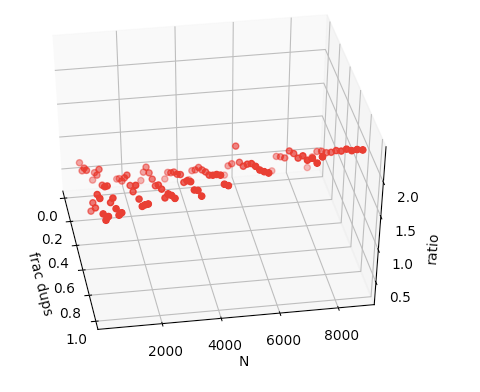What we can see is that the ratio variable increases almost linearly with N and frac. Almost linearly. At this point, one idea is to compute both the statistics (N, frac) for a given dataset (at runtime, it's cheap), and predict what its ratio is going to be. If that value is above 1, use single algorithm, else use batch algorithm.

We can run a simple linear regression against the dataset of runtimes, and we get the following:

import statsmodels.api as sm
X = results[["N", "frac"]]
Y = results["ratio"]
model = sm.OLS(Y, X).fit()
print(model.summary())
OLS Regression Results
==============================================================================
Dep. Variable:                  ratio   R-squared:                       0.933
Method:                 Least Squares   F-statistic:                     725.8
Date:                Thu, 03 Jan 2019   Prob (F-statistic):           3.34e-62
Time:                        13:11:37   Log-Likelihood:                 68.819
No. Observations:                 108   AIC:                            -131.6
Df Residuals:                     105   BIC:                            -123.6
Df Model:                           2
Covariance Type:            nonrobust
==============================================================================
coef    std err          t      P>|t|      [0.025      0.975]
------------------------------------------------------------------------------
const          0.3396      0.030     11.367      0.000       0.280       0.399
N           4.135e-05   4.63e-06      8.922      0.000    3.22e-05    5.05e-05
frac           1.5038      0.041     37.039      0.000       1.423       1.584
==============================================================================
Omnibus:                        3.371   Durbin-Watson:                   1.064
Prob(Omnibus):                  0.185   Jarque-Bera (JB):                3.284
Skew:                          -0.166   Prob(JB):                        0.194
Kurtosis:                       3.787   Cond. No.                     1.77e+04
==============================================================================

Plotting this plane-of-best-fit:We can see that the fit is pretty good, but there is some non-linearities in the second figure we aren't capturing. We should expect non-linearities too: in the batch algorithm, the average batch size is (N * frac) data points, so this interaction should be a factor in the batch algorithm's performance. Let's include that interaction in our regression:

import statsmodels.api as sm
results["N * frac"] = results["N"] * results["frac"]
X = results[["N", "frac", "N * frac"]]
Y = results["ratio"]
model = sm.OLS(Y, X).fit()
print(model.summary())
OLS Regression Results
==============================================================================
Dep. Variable:                  ratio   R-squared:                       0.965
Method:                 Least Squares   F-statistic:                     944.4
Date:                Thu, 03 Jan 2019   Prob (F-statistic):           2.89e-75
Time:                        13:16:48   Log-Likelihood:                 103.62
No. Observations:                 108   AIC:                            -199.2
Df Residuals:                     104   BIC:                            -188.5
Df Model:                           3
Covariance Type:            nonrobust
==============================================================================
coef    std err          t      P>|t|      [0.025      0.975]
------------------------------------------------------------------------------
const          0.5465      0.030     17.941      0.000       0.486       0.607
N          -1.187e-05   6.44e-06     -1.843      0.068   -2.46e-05    9.05e-07
frac           1.0899      0.052     21.003      0.000       0.987       1.193
N * frac       0.0001    1.1e-05      9.702      0.000    8.47e-05       0.000
==============================================================================
Omnibus:                       10.775   Durbin-Watson:                   1.541
Prob(Omnibus):                  0.005   Jarque-Bera (JB):               21.809
Skew:                          -0.305   Prob(JB):                     1.84e-05
Kurtosis:                       5.115   Cond. No.                     3.43e+04
==============================================================================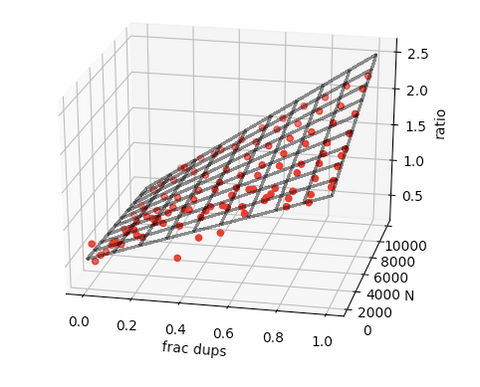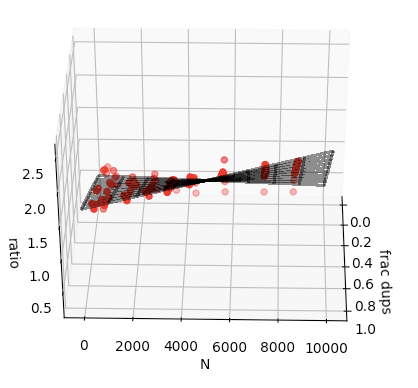Looks like we capture more of the variance ($$R^2$$), and the optical-fit looks better too! So where are we?

1. Given a dataset, I can compute its statistics, N and frac, at runtime.
2. I enter this into my linear model (with an interaction term), and it predicts the ratio of batch performance to single performance
3. If this prediction is greater than 1.0, I choose single, else batch.

This idea was recently implemented in lifelines, and with some other optimizations to the batch algorithm, we see a 60% speedup on some datasets!

Notes and extensions

• This is a binary problem, batch vs single, so why not use logistic regression? Frank Harrell, one of the greatest statisticians, would not be happy with that. He advocates against the idea of false dichotomies in statistics (ex: rate > some arbitrary threshold, unnecessary binning of continuous variables, etc.). This is a case of that: we should be modelling the ratio and not the sign. In doing so, I retain maximum information for the algorithm to use.
• More than 2 algorithms to choose from? Instead of modelling the ratio, I can model the performance per algorithm, and choose the algorithm that is associated with the smallest prediction.
• L1, L2-Penalizers? Cross-validation? IDGAF. These give me minimal gains. Adding the interaction term was borderline going overboard.
• There is an asymmetric cost of being wrong I would like to model. Choosing the batch algorithm incorrectly could have much worse consequences on performance than if I chose the single algorithm incorrectly. To model this, instead of using a linear model with squared loss, I could use a quantile regression model with asymmetric loss.

¹ ugh, naming is hard.

]]>
https://dataorigami.net/blogs/napkin-folding/two-ways-to-measure-heroes-of-the-storm-win-rates 2018-09-13T22:31:00-04:00 2018-09-14T11:46:59-04:00 Causal Inference & Heroes of the Storm Win Rates Cameron Davidson-Pilon

I've been playing the video game Heroes of the Storm by Blizzard for about 3 years now. I even attended the game's professional scene's championship last year! One part of the game that has attracted me is the constantly accumulating character roster (I'll provide a short summary of the game later in this post). This means that new characters are being added, and existing characters are being tweaked if they are too powerful or not powerful enough. It's this latter feature that I am most interested in, and the purpose of this blog post: how do we know when a character is over or under powered? There are two methods: a wrong method, and a less-wrong method. This blog article's goal is to re-describe the the old, wrong method, and propose a new ideas for character balance. Keep in mind: the estimated values count for less than the description of the idea.

But before we get into the methods, a quick summary of Heroes of the Storm gameplay (you can skip if you are already familiar).

Gameplay

Heroes of the Storm is a 5-player vs 5-player team game. Before the game begins, each player choose a unique character (called heroes in the game) and is combined with four other players to battle against the opposing team. The objective is to destroy the opponents "core", using either the characters' unique abilities or powerful bonuses scattered around the play area (called a "map"). The game ends when either team's core is destroyed.At the time of writing, there are over 70 characters, each with unique abilities and advantages. A players decision to choose a characters is based on i) their affinity for a character, ii) which characters are strategically advantageous, given what your opponent is playing (called the "meta"), or iii) which characters are over powered. Focusing on this last reason, a character can be over powered / under powered if their abilities are providing too much damage, healing, etc. Blizzard, the game's creator, will address these different power level of characters with weekly balance patches - however with the game constantly changing, there will never really be perfect equality.

Statistics of the game

Players can upload their games to third-party services, which aggregate all the games players, characters, map and outcomes into valuable statistics. A very influential statistic is the win rate of character i, defined as:

$$\frac{\text{wins}_{i}}{\text{games participated}_i}$$

which is an estimator for:

$$P(\text{win} \;|\; \text{i present in game})$$

Ideally, the win rate of all characters are close to 0.5, as 0.5 is suppose to represent a character that is equally like to win as to lose (and hence "balanced"). Estimates typically range from 0.35 for the most under-powered, to 0.65 for the most over-powered. In fact, the ordering of this estimate over all characters is commonly used by players to find the most over-powered and under-powered characters, and call for "nerfs" or "buffs" respectively to rebalance the character.

But this is wrong. The win rate is only a correlation. It's not taking into account the entire causal picture. Let's explore that next.

The confounder problem

The problem with the above estimate, which I call the naive win rate, is that character selection and the chance of winning have common causes. These common causes are called confounders. For example, professional players may a) select certain characters more often than others and b) win more often. In an extreme case, if the best player in the world selected character T almost exclusively, then character T would have a higher than average win rate, but not necessarily due to the character.

Another example: depending on the other characters on an allied team, a) selection of a character changes, and b) probability of winning changes. If character T is often paired together with S because of some pair synergy, then the win rate of T will be influenced by the win rate of S.

Another example: the higher a team's average MMR (a measure of experience) may a) select a "meta" character more often and b) win more often. More specifically, the more experienced a team, the more likely they will recognise the best character to play in response to their opponents character, and because of such experience, are more likely to win the game.

Another example: when a new hero is released, or an existing hero gets major changes, less experienced players may choose them more. This has an effect on character selection, and on the win rate. Another confounder.

The point is: there exist many causes of both character selection and win probabilities. This means that the naive win rate is not necessarily a good measure of balance, but simply a correlation (not causation) between character selection and winning.

In fact, if you are familiar with causal diagrams, this is perhaps what the causal diagram looks like:

The arrow we are interested in is the arrow between character and win, which I call the causal win rate. The strength of this arrow (difference from 0), and its direction (positive or negative) tell us if a character is over or under powered. This tells us "how much more likely is a win if you choose character T instead of character S?"

Controlling confounders

We have lots of statistical methods to handle the confounders that muddy our naive win rate. The end result is an estimate of the causal win rate. However, causal inference requires you to state assumptions. Here's the big causal assumption, called exchangeability:

Controlling for the map, opponents' average experience, opponents' characters, allied characters, and allied experience, the win rate of those players that did and didn't choose character T would be the same on average if they all chose character T.

We are also going to simplify the problem, which I'm sure is wrong but it's a first approximation. Let's assume that the character in question is chosen last. That is, the decision to choose character T happens with perfect information of all other character selections and map knowledge. We are also assuming no effect modification (which certainly exists). Effect modification is when the effect (causal win rate) can be modified by other factors. For example, we already mentioned above that possible synergies can exists between pairs of characters - that is a form of effect modification. Similarly, the team composition (ex: how many healers the team has, how many damage dealers, etc.) is a form of effect modification. Like I said, we are going to ignore effect modification for now, and will return to it in another blog post.

For our modelling, we will use outcome regression, which is a causal inference method that carries with it lots of modelling assumptions (Note: there are better methods which have less model assumptions). For example: we are assuming a linear relationship between outcome and variables and we are assuming no interactions (effect modifiers).

Our regression will look like:

\begin{align} \text{logit}(\text{win}_i) & = \alpha_1 \text{AlliedVallaPresent}_i \\ & + \alpha_2 \text{AlliedLiLiPresent}_i + ... \\ & + \beta_1\text{AvgAlliedMMR}_i \\ & + \beta_2\text{AvgOppMMR}_i \\ & + \beta_3\text{Map}_i + ... \\ \end{align}

where each a coefficient represents a scalar or vector (as in the case of $$\text{Map}_i$$).

Note that (and this is a consequence of our simple model) that all characters in the regression are symmetric. Thus we just need to run a single regression, and the parameters $$\alpha_i$$ are all we are interested in.

Interpretation

What does $$\alpha_i$$ represent though? Nothing without a reference point. I chose to use the character Kerrigan as the reference character. A positive alpha represents a better causal win rate relative to Kerrigan, a null alpha represents an equal causal win rate relative to Kerrigan, and a negative alpha represents a worse causal win rate relative to Kerrigan. These relative comparisons may seem like a disadvantage, but I chose Kerrigan specifically as she was the median character with respect to causal win rate (so I ran the program once, using some other character, and Kerrigan was the median in that analysis). Truthfully, it doesn't really matter who is the reference character, the ordering between characters is agnostic to the reference character. However, I choose the median character because they create a natural division of who is over-powered and who is under-powered.

Dataset

The dataset we are going to use is from user-submitted games to hotslogs.com. The time period is 4/26/2017 to 5/9/2017 which is a period inbetween two balance patches. I filtered down to Hero League and Team League games, and sampled 50k games for analysis.

Results

After we fit the regression, we have an estimated value of alpha for each character. Importantly, we also have error estimates for each alpha that we use to form confidence intervals for each alpha. If this confidence interval contains 0, we can conclude that the character is either balanced, or unbalanced too little to detect. Here are the results (clickthrough for a larger image):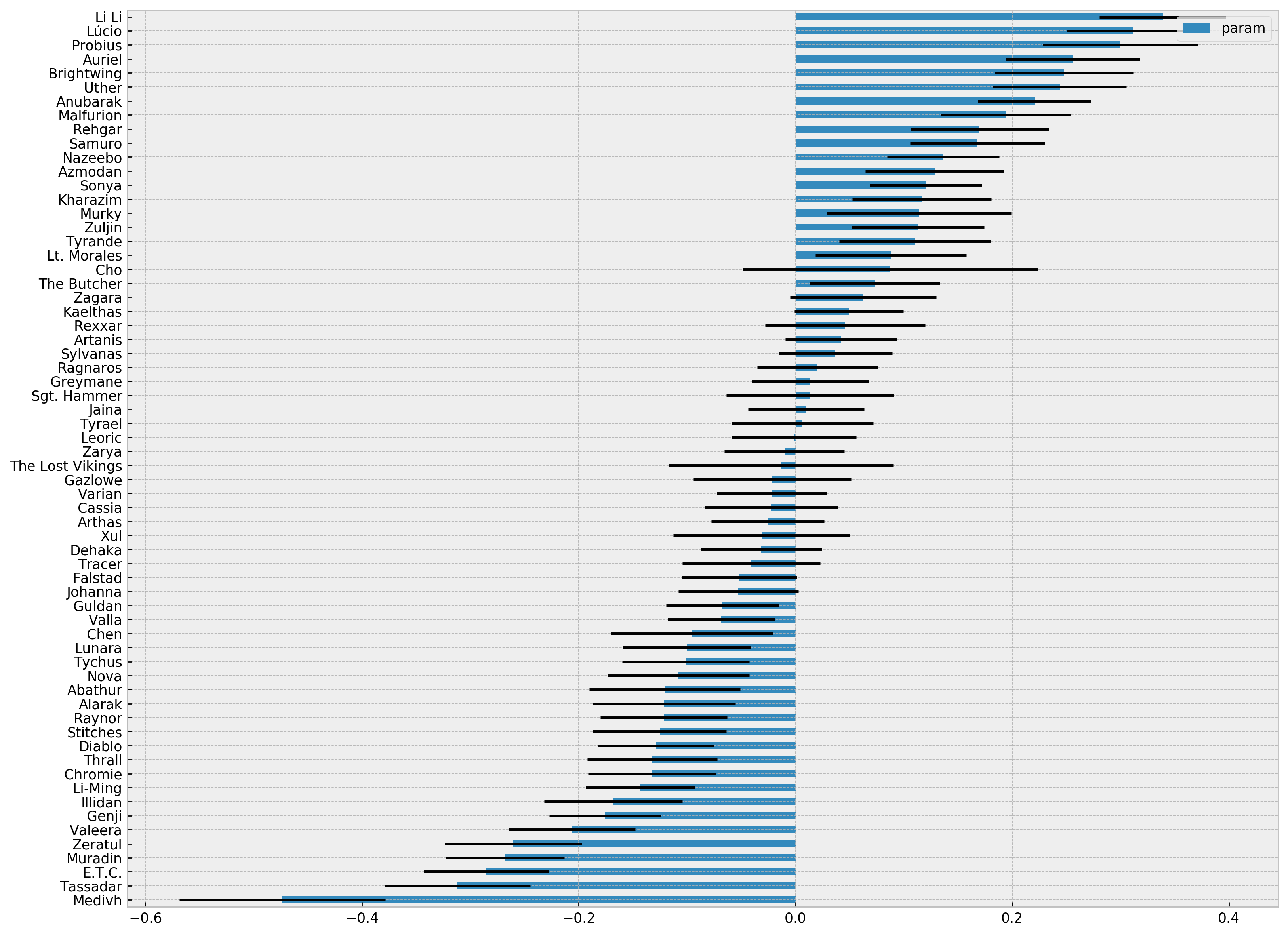The characters Li Li, Lucio, and Probius are the strongest. On the other hand, Medivh, Tassadar and E.T.C. are the weakest. For some evidence that our analysis is not absolutely wrong: 5 of the top 6 characters above were nerfed in the May 10th, 2017 balance patch.

How does our causal win rate estimates compare to the naive win rate?(Clickthrough for a larger image)

The two metrics are positively correlated, which offers evidence that the naive win rate isn't biased too much by the confounders. The characters in the upper-left and lower-right quadrants are characters that the community is probably thinking about wrong. For example, Lt. Morales has a low naive win rate, and the community might suggest she get a buff. However, she likely is over performing in this dataset. Another very interesting thing to point out is that all the support characters are "shifted" about 0.2 units to the right. Historically this period was when the double-support meta (two or more support characters per team) was gaining popularity, perhaps because Blizzard kept supports artificially over powered as the above graph suggests. Note that this support character advantage is hidden if we only looked at naive win rates. This support advantage is not a artefact of the analysis either, as when I did the same analysis for a more recent dataset, the support shift wasn't present any more.

More recent data, and the effect of balance patches

I managed to get a more recent data (as of the time of writing) from July 2018, spanning a balance patch. So we can observe what happens to heroes' win rates as they were buffed or nerfed on the July 25th balance patch. First, some of the nerfed characters:

• Raynor was severely nerfed in this balance patch, and this is reflected in his pre and post win rates.
• Deckard Cain and Yrel were nerfed.
• Cho, famously, lost his unstoppable on Surging Dash. This significantly lowered his win rates. This is much more clear when we look at his causal win rate and not his naive win rate.

And buffs: Azmodan and Kael'Thas saw straight buffs, however Hanzo saw some buffs and some nerfs, and his causal win rate stayed about the same.

Conclusion

• I wasn't able to model a player's MMR, and instead used a team's average MMR, which will be less accurate. Consider this a form of measurement error.
• Excluding effect modification is a serious flaw. Modelling effect modification would not only give us better estimates, but also aid in finding synergies and anti-synergies in team compositions & maps.
• My model assumed a linear relationship between all variables and the outcome. Though I did include some quadratic terms for the average MMR of teams, there is likely still lots of model misspecification bias occurring.

What I hope from this article is that Heroes of the Storm players, and players of similar games, rethink how the naive win rate can be biased. However, one thing that I'd like to think about more is how biased are we? We saw that there was a high correlation between the naive and the causal win rate - does this imply we can use the naive win rate in most circumstances? I'm not sure, and it's something I want to explore. An approach is to use a causal inference model with less assumptions, and including effect modification, to see if we still see a high correlation between the two rates.

Sketch code is available on my Github here.

]]>
https://dataorigami.net/blogs/napkin-folding/everything-i-need-to-know-about-causality-learned-smoking 2018-08-25T18:54:00-04:00 2019-03-12T11:17:46-04:00 Everything I need to know about causality, I learned smoking cigarettes Cameron Davidson-Pilon I've gone through a causal inference revolution, and I can't go back. Causal inference is all I think about now, but unfortunately its importance is often overlooked. Part of my revolution came after reading Miguel Hernán et al's paper on infant mortality and smoking, The Birth Weight "Paradox" Uncovered?. It took me a full evening to really understand its points, and it was rewarding when I finally did. In this blog post, I'm going to examine the paper from my point of view, and add or expand on details that I wish the original authors had. In writing this blog post, I have two goals in mind. The first is to solidify the ideas in my head. No better way to do that than to have to explain it to others. The second goal is to provide a shortcut for others to understand why causal inference is important.

Introduction

The authors start by saying that researchers will naively stratify a risk factor, like smoking, by the birthweight (BW) of infants. Researchers noticed that if the risk factor produced low birthweight (LBW) infant more often, then there would be a specific birthweight where infants below this threshold would have a lower mortality if in the riskier group. For example, for infants born below ~2000g, those infants who were exposed to maternal smoking actually had a lower infant mortality rate than those not exposed to maternal smoking. See figure below:This seems like madness - not smoking is associated with higher infant mortality in some populations! Some researchers have even suggested that:

"the effect of maternal smoking is modified by birth weight in such a way that smoking is beneficial for LBW babies."

Let's investigate what is really going on.

Material and Methods

The authors use a large dataset of over 3 million infants. They define LBW as less than 2500g. Their metric of interest, infant mortality, is defined as a death in the first year per 100,000 livebirths. How do they summarise the effect of maternal smoking?

"We used logistic regression to estimate infant mortality rate ratios and 95 percent confidence intervals..."

In other words, they will be using a specific coefficient in a logistic regression model to produce a summary of the effect of maternal smoking on mortality. Specifically, if we let $$M$$ denote the presence of maternal smoking (0 or 1) our model might look like:

$$\text{logit}(p_i) = \beta_0 + \beta_1 M_i$$

and so $$\beta_1$$ summarizes the effect of maternal smoking. We'll call the above model the "crude" model. Let $$B$$ denote birthweight. We call the following model the "adjusted" model:

$$\text{logit}(p_i) = \beta_0 + \beta_1 M_i + \beta_2 B_i$$

The next question to ask is what else to control for in this regression. The authors say:

"control for potential confounders (maternal age, gravidity, education, marital status, race/ethnicity, and prenatal care). Since adjustment for these potential confounders had virtually no effect, we present only the unadjusted rate ratios below."

What does this mean? If we extended the model to:

$$\text{logit}(p_i) = \beta_0 + \beta_1 M_i + \beta_2 B_i + \beta \cdot C_i$$

where $$C$$ are other controls mentioned above, this doesn't change the conclusion (or the coefficients - it's not clear), so the author choose to present results without the additional controls.

Naive Analysis Results

After running the regression, the crude model produced that $$\beta_1 = 0.438 \pm 0.029$$. (The paper actually uses the rate ratio, which is the exponential of the coefficient in the model - we won't). However, if we use the adjusted model, then $$\beta_1 = 0.049 \pm 0.032$$, a 10-fold decrease. What happened? Why, after conditioning on BW, did we seem to lose so much effect? It gets worse, in fact.

If we stratify the population into LBW and non-LBW (a "stricter" way to control for a continuous variable), and train the crude model on both these sub-group, we see that smoking actually benefits the infants in the LBW sub-group: the rate ratio of maternal smoking less than 1 (the opposite conclusion is found for the non-LBW sub-group).

Possible causal diagrams

I'm going to assume the reader is familiar with causal diagrams. I really liked that the authors went through increasingly complex causal diagrams to explain the paradox. I'll do the same. (Diagrams produced by my latest side project, dog).

Diagram 1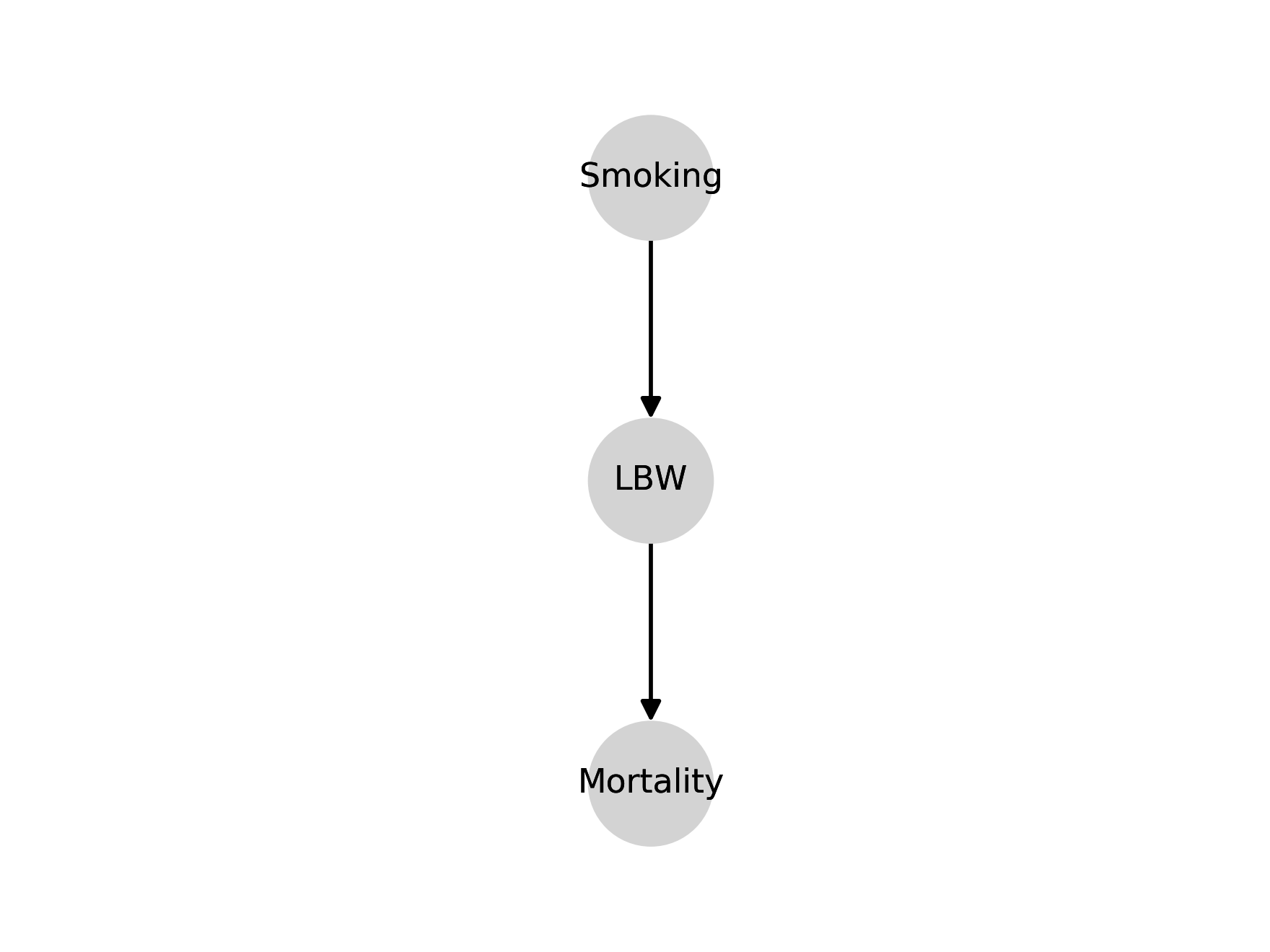This is the simplest model of our causal relationships. Smoking causes LBW, but has no direct effect on mortality, except through LBW. A very useful thing I learned from this paper is that a causal diagram has implications that the data should support, and if the data doesn't support it, the causal diagram is invalidated! Let's see how this works using the results from the regressions. If the above causal diagram is true, then smoking and mortality are certainly correlated. And we see this in the crude regression above (the coefficient is not 0). On the other hand, this diagram also implies that conditioning on BW means that smoking and mortality are independent. However, in the adjusted model above, where we do condition for BW, we still see that the coefficient of smoking is still far from 0. Thus, the data suggests that smoking and mortality are dependent, conditioned on BW. Hence, this diagram is wrong.

Diagram 2We had success with our implication test above. Let's try the same test on this new causal diagram. In the above causal diagram, if we condition on smoking, LBW and mortality should be independent. However, in the adjusted model, LBW has a non-zero coefficient. So we can refute the above diagram.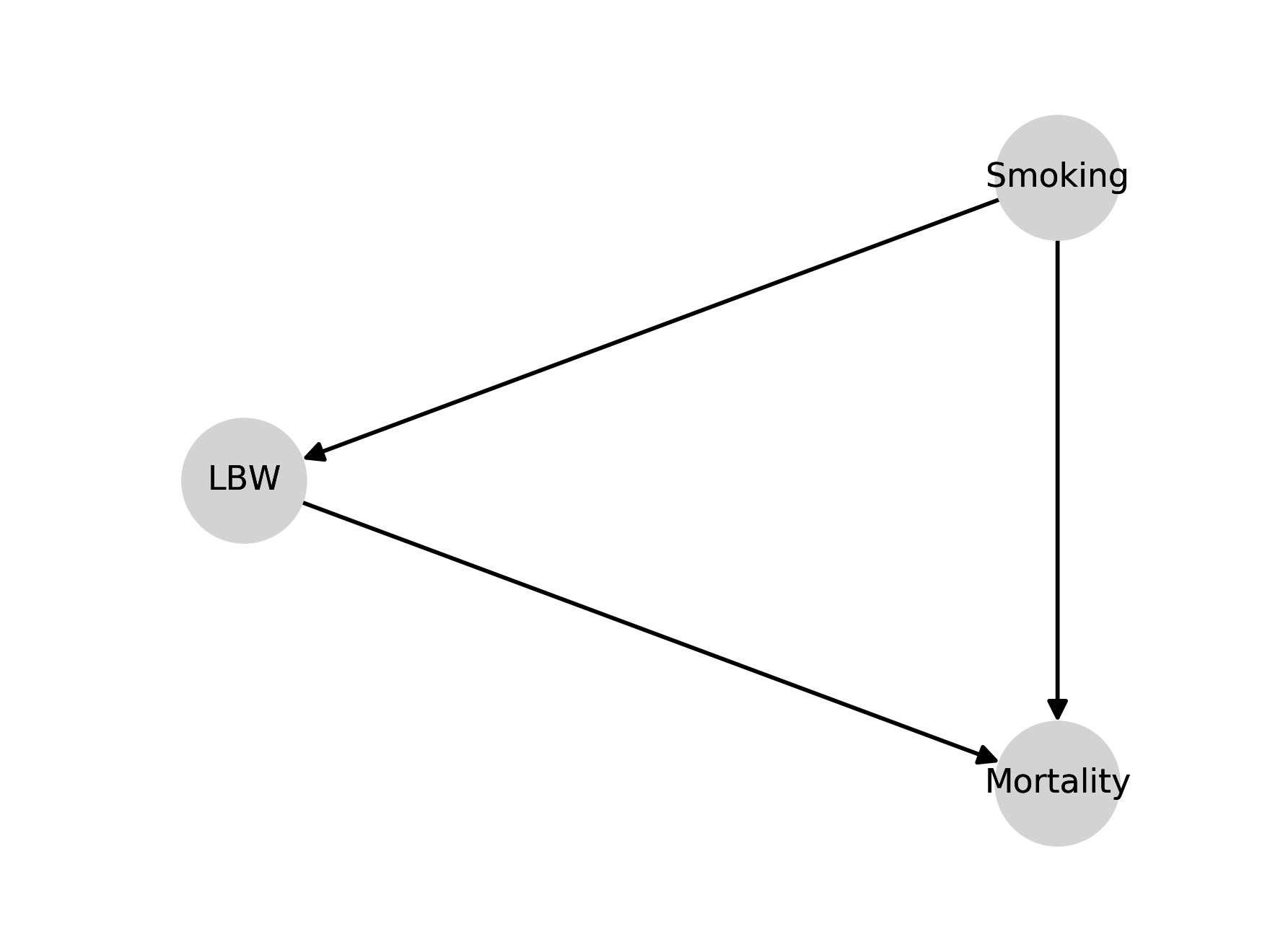In this new model, we have smoking causes LBW, smoking causes mortality and there is a causal relationship between LBW and mortality. We see that LBW is a mediator for smoking. The authors are more precise and say that smoking is partially mediated through LBW, since there still is a direct effect of smoking on mortality. The authors state that this DAG is actually the simplest model, because it is "complete" (I suppose they mean this in the graph-theory sense of "complete"). In other words, the previous two graphs had very strict constraints on relationships that allowed us to "test" the graphs. The above graph doesn't have this property, and technically has the least amount structure. However, it probably presents the most accurate model of reality thus far.

This is where the story gets interesting. If you showed this model to domain experts, they would say it is too simple. A domain expert would suggest that there are other, possibly unobserved, factors involved in BW, like birth defect. If this is true, bad things can happen. Let's explore this in diagram 4. (From here, I'll actually diverge a bit from the paper, but only because the paper does it's presentation of diagrams in an odd manner.)

Diagram 4This new diagram introduces an unobserved variable (in blue), birth defects, and removes some causal links - but as we'll see even this simple diagram is enough to give us our "paradox". Birth defects also causes LBW and also has a (strong) positive effect on mortality. So, given this diagram, let's see what happens when we condition on LBW. Among LBW infants, if the mother didn't smoke, then we know the only other cause for LBW was birth defects. By conditioning on LBW, we have introduced a spurious correlation between smoking and birth defects ("if not this, than that") - in fact, this association is negative. And because birth defects has a positive association with mortality (and smoking has none), we have introduced a negative association between smoking and mortality.

The problem occurs because birth defects is unobserved. If we had observed birth defects, we could have controlled for it and everything would have been fine.

The story above doesn't mention observations - so why is observation so important? Even though we don't observe birth defects, the "effect" of it is still present in the outcomes. And because BW is caused by it, the effect of birth defects is passed through to BW in any analysis. This means that even though BW has no effect on the outcome, it will still look like it because it's highly correlated with birth defects.

Diagram 5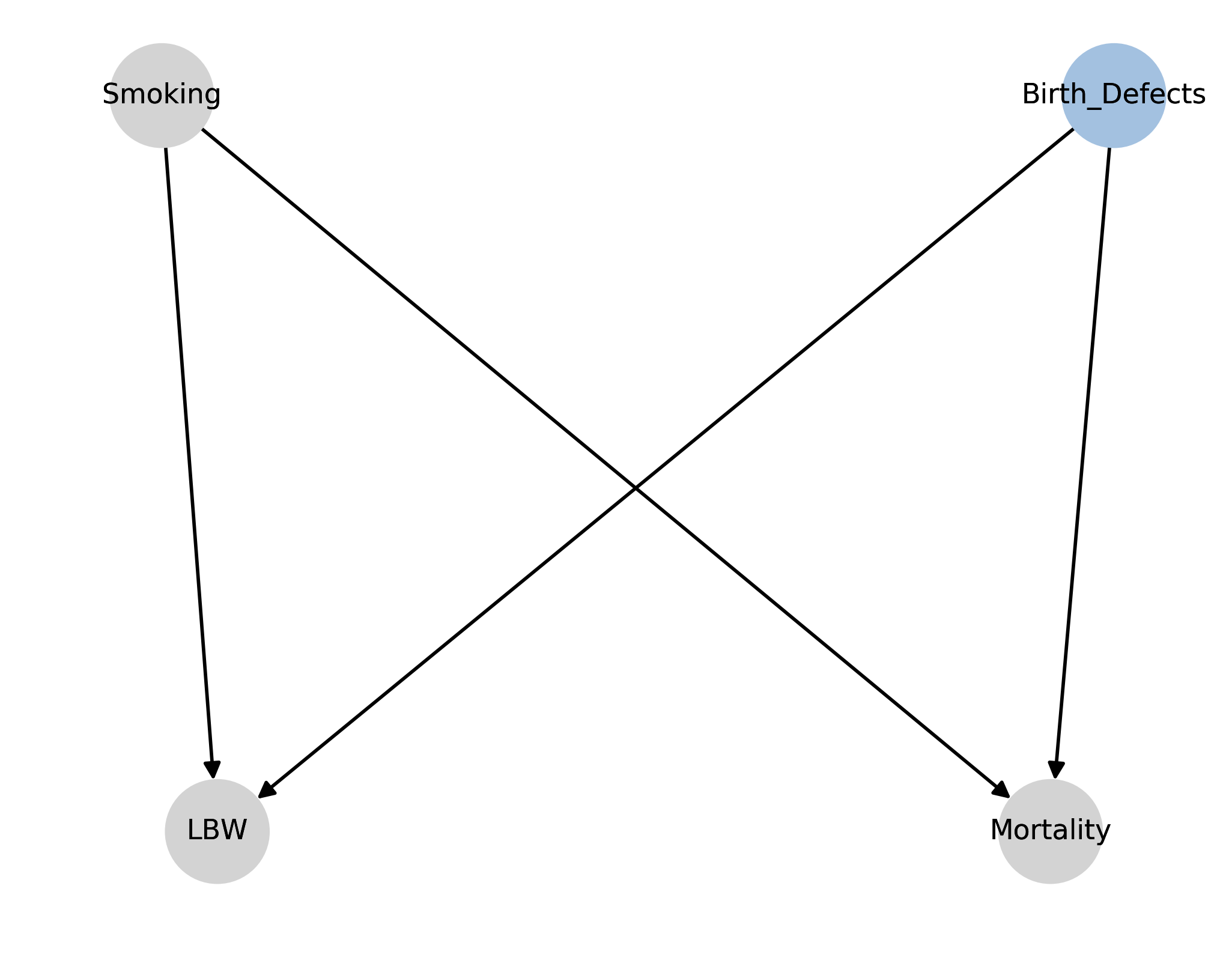Let's introduce a link between smoking and mortality now. The authors state

In this case, the rate ratio adjusted for birth weight would be a combination of 1) the true direct effect of smoking on mortality and 2) the bias resulting from the presence of common causes of LBW and mortality.

It's not obvious which effect is larger in magnitude, apriori. Similar to above, if we could control for birth defects, this bias would vanish.

Diagram 6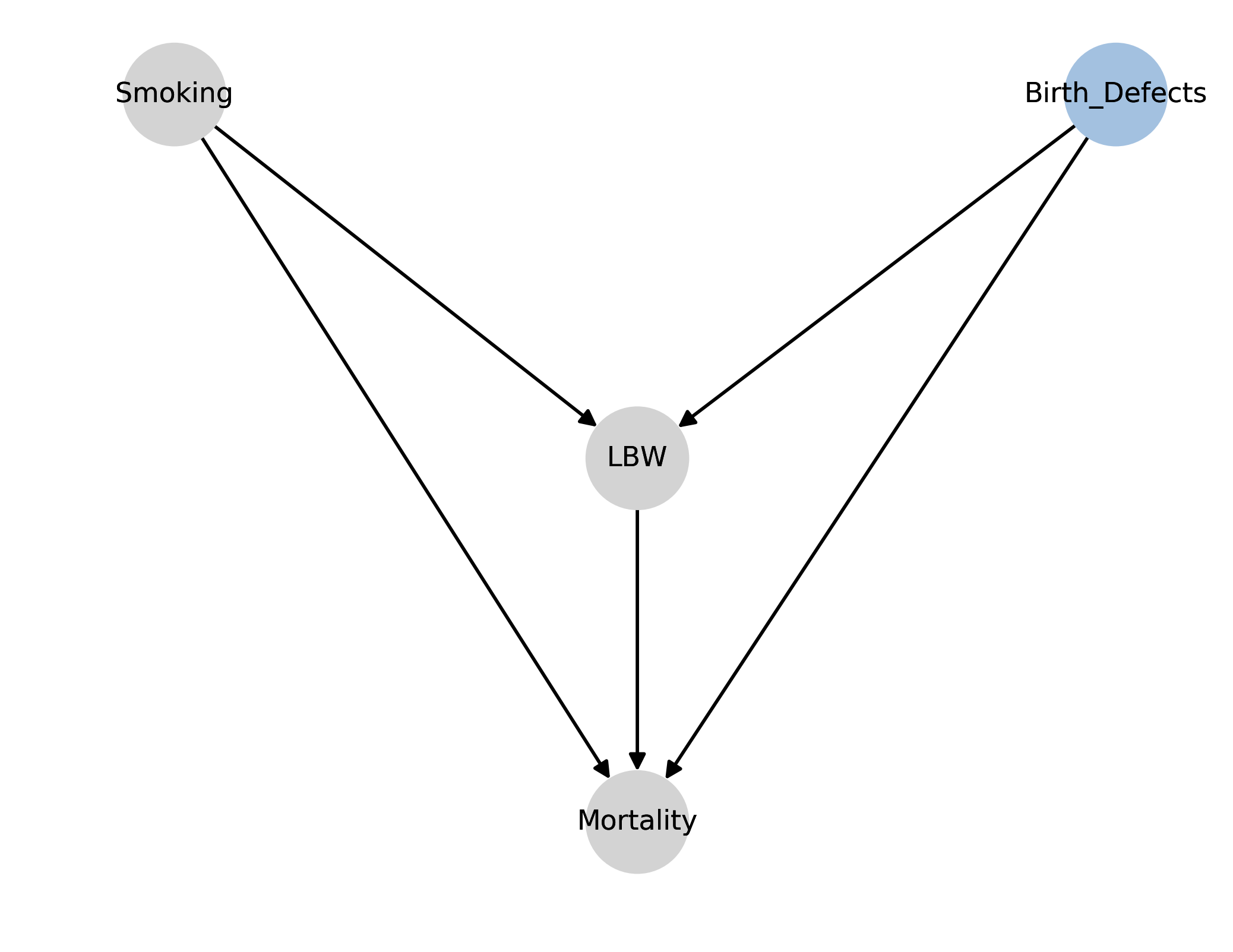With this diagram, we've added a link between LBW and mortality, adding more plausibility to our diagram. The same bias still remains, however.

Diagram 7The authors then put forward another diagram that suggests that only some types of low birth weight (LBW_B) are actually harmful. The argument is as follows.

Some instances of LBW are not harmful, like being born in a higher altitude. That is, LBW caused by higher altitude infants do not see a higher risk than their counterfactual counterparts born at normal BW in a low altitude area. This LBW is of the type LBW_A. However, a LBW in a low altitude area is of much higher risk, since the cause of the their LBW isn't LBW_A but LBW_B, and is of much higher risk. In this case, if we condition on LBW, we would introduce the same biases observed above.

Discussion

This is probably my favourite part of the paper. The authors ask "why are we conditioning on BW anyways?" Researchers can be interested in two questions: what is the total effect of smoking, and what is the direct effect of smoking (aside: I learned a lot about the differences between total and direct effects by reading about the Table 2 fallacy.) If the researchers' goal is compute the total effect of smoking on mortality, then adjustment for a child variable (like BW) is not correct. (The author's state that, for many sensible reasons, BW is a cause of smoking, and not vice versa). In fact, the only adjustment needed is for confounders of smoking and mortality. This, according to the back-door criteria, is sufficient to compute the total adjustment.

However, if the researchers goal is to compute the direct effect, things are more difficult. To compute a direct effect, one needs to control for any mediators of smoking. This makes controlling for BW sensible. But then, we need to make sure that if those mediators are colliders (have more than a single cause), we need to control for all their parents too to satisfy the backdoor criteria. This is what happened in Diagram 4 above: because we conditioned on BW, but failed to condition on unobserved birth defects, our estimate of the direct effect of smoking was biased.

In reality, I imagine the original researchers did not have a causal diagram in mind when they first encountered the paradox. I doubt they even had the vocabulary of direct or total effects.

In conclusion, I hope this articled helped understand this highly important and influential paper. Apriori, we know that smoking is bad (hence the confusing "paradox"), but how often might this happen in less conspicuous contexts? Without explicitly drawing or stating a causal diagram, it's easy to make the researchers' original mistake and think some interesting phenomenon is occurring.

]]>
https://dataorigami.net/blogs/napkin-folding/three-pillars-of-data-science 2018-06-20T14:47:00-04:00 2018-06-24T13:11:56-04:00 Three Pillars of Data Science Cameron Davidson-Pilon The idea of grouping data science tasks has been bouncing around in my head for the past six or so months, and recently I came across a paper by Miguel Hernán¹ that summarised the ideas nicely. In fact, the metaphorical "pillars" idea came from his paper. This blog article is a combination of his ideas and my own. With that, I present what I (and he) consider the three pillars of data science.

More

]]>

Introduction

The idea of grouping data science tasks has been bouncing around in my head for the past six or so months, and recently I came across a paper by Miguel Hernán¹ that summarised the ideas nicely. In fact, the metaphorical "pillars" idea came from his paper. This blog article is a combination of his ideas and my own. With that, I present what I (and he) consider the three pillars of data science.

Description

Description is what I feel the majority of data scientists in industry focus on. This could be something as simple as mean, a conversion rate, a time series, etc. or something more advanced like a statistical test. Description also includes data mining algorithms like clustering and association rules.

This is a well explored area, thanks to the 20th century focus on descriptive statistics and methods. This was the result of a few simultaneous conditions:

1. Due to the lack of computers and often inability to share datasets, statisticians and scientists needed ways to summarise datasets.
2. Hand in hand with advances in healthcare and agriculture were ways to understand the effectiveness of these advancements. With not only finite but small sample sizes, and lives on the line, it was of paramount of importance to measure the variance and potential errors.
3. Towards the end of the century, when computers become more available, new methods become available too: GLMs, bootstrapping, data mining.

While this is what I feel is the most common pillar in industry, it's not talked much about in the community. I wonder if is this because it's due to the classical feel of these techniques? Or perhaps it's what I would prefer but do not believe, is that they are so well studied and understand that we need not talk about them. (Why do I not believe this? Take a look at Andrew Gelman's terrific blog almost any time he posts an article. It's always full of mistakes other researchers make, let alone people in industry). No, most of the community's attention is given to the next pillar: prediction.

Prediction

We spend most of our time thinking, implementing, watching and talking about prediction. Many times a day, a new ML prediction algorithm or article will surface on Hacker News. Contrast where we are today with the previous century: we have lots of compute power, available datasets and accessible technology for prediction. Prediction, in some cases, has been widely profitable for companies. (Not to say description hasn't either. Pharma companies rely on descriptive methods to validate their work. Banks and insurance companies, too, rely on descriptive methods to control their business investments - no other technique could help.)  I say some cases, because I do see a lot of excess application of prediction, often where description (or the third pillar) would be sufficient.

The next pillar is the least talked about. Surprisingly too, considering the importance of it.

Causal Inference

Sometimes I feel ashamed to admit how ignorant I really am. To be transparent, I didn't learn much causal inference (or care) until about nine months ago. And even then, it was just fumbling around in a dark room of ideas. Slowly, however, I picked up the pieces and learned what to learn. So why should a data scientist know about causal inference?

From my experience, a data scientist gets asked questions that can only be answered with a causal inference lens. Questions like, to use my company Shopify as an example:

1. What is the impact on sales of merchants who adopt our point-of-sale system?
2. What is causing merchants to leave our platform?
3. What is the effect of server latency on checkouts?

These questions, because of the complex nature of the system, cannot be answered with traditional summary statistics. Confounders abound, and populations are biased. We require new tools and ideas that come from causal inference.

Randomised control trials (RCTs) are very common in tech companies. This classical technique is actually generalised in causal inference frameworks. Beyond this, as a data scientist working in industry, you'll have a new perspective on what your observational data can (and cannot!) deliver.

What I find worrying is that, as a community, we are not talking about causal inference enough. This could have some serious harm. If practitioners in industry aren't aware of techniques from causal inference, they will use the wrong tool and could come to the wrong conclusion. For example, both Simpson's Paradox and Lord's Paradox, two very common phenomena, are easy explainable in a causal framework, but without this framework, people can make disastrous and harmful conclusions. For example, in the low birthweight paradox (see paper below), without causal inference there is the potential to conclude that smoking helps low birthweight infants! Furthermore, I rarely see school programs or bootcamps in data science teaching causal inference. It's almost exclusively prediction and technology!

So, rather than describe the slow and meandering way I discovered causal inference, I'll post some links below to give you a head start (probably read these in order):

There's so much more, but at least this gives you a place to learn what to learn﻿.

Conclusion

This article will likely evolve over time, as I discover more and more about the boundaries of these three pillars. But I hope the reader recognises a pillar they are lacking in (for me it was causal inference). Based on this construction, I do expect there to be huge potential for practitioners of causal inference (ex: econometricians and epidemiologists come to mind) in teaching data science.

]]>
https://dataorigami.net/blogs/napkin-folding/how-shopify-merchants-can-measure-retention-x-post-from-shopify-engineering-blog 2017-11-25T10:54:00-05:00 2017-11-25T10:54:52-05:00 How Shopify Merchants Can Measure Retention [x-post from Shopify Engineering Blog] Cameron Davidson-Pilon How Shopify Merchants Can Measure Retention]]> https://dataorigami.net/blogs/napkin-folding/a-self-describing-sequence-problem 2017-08-19T13:41:00-04:00 2017-08-20T16:41:47-04:00 A self-describing sequence problem Cameron Davidson-Pilon Each week FiveThirtyEight posts a mathematical riddle to solve over the weekend. This latest week's problem was interesting, and I wanted to post my solution. The original problem is:

Take a look at this string of numbers:

333 2 333 2 333 2 33 2 333 2 333 2 333 2 33 2 333 2 333 2 …

At first it looks like someone fell asleep on a keyboard. But there’s an inner logic to the sequence: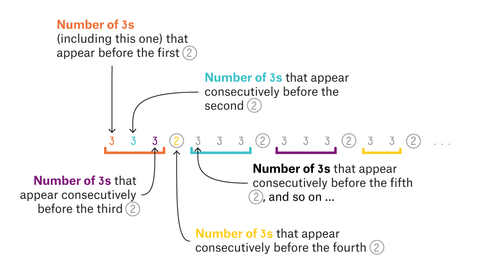This sequence creates itself, number by number, as explained in the image above. Each digit refers to the number of consecutive 3s before a certain 2 appears. Specifically, the first digit refers to the number of consecutive 3s that appear before the first 2, the second digit refers to the number of 3s that appear consecutively before the second 2, and so on toward infinity.

The sequence never ends, but that won’t stop us from asking us questions about it. What is the ratio of 3s to 2s in the entire sequence?

Let's first implement the sequence in Python. We can use a queue to hold the sequence, and when we pop a new element off the queue, we can extend the queue (adding three 3s and a 2, or two 3s and a 2) based on the value of the popped element.

We could brute force the solution by computing the ratio as we extend the sequence ever longer, but this will only ever give us an approximation, and no realisation as to why the solution is so.

I'm going to show off how I played around with the problem, naively, and then started to formulate a solution. The first thing I computed was the ratio of 2s present in the sequence. This isn't the desired answer (ratio of 3s over 2s), but the ratio of 2s, which i'll call $$R$$, can be used to find the original solution too: $$\frac{1- R}{R}$$. Also, $$R$$ can be seen as a probability, something that didn't turn out to be useful but was a possible attack. Using the Python code, R was computed to be approximately 0.2679491. So I had some place to start.

Next I looked at where the 2s were placed in the sequence. Ex: position 4, 8, 12, 15, and so on were 2s. Extending, 2s were placed at positions:

4, 8, 12, 15, 19, 23, 27, 30, 34, 38, 42, 45, 49, 53, 56, 60, 64, 68, ...

Inspecting this, it's clear that 2s will occur every fourth number, except every 4th time, when it appears after three numbers. Call this last exception a "skip". Playing around with this pattern, I constructed a crude function to estimate the position of the $$n$$th 2, $$4n - 4n / (16)$$. (I didn't simplify this to make it more clear: the first term is where I expect the position to be given there were no "skips"; the second term is the estimated number of skips after $$4n$$ elements of the sequence)

It gave pretty close results for values of $$n$$ under 50. I could also estimate $$R$$ by looking at $$\frac{n}{4n - 4 n / 16}$$. For large $$n$$, this gave a number pretty close to my Python approximation of $$R$$ (above). But it wasn't close enough, and something was wrong. Simplifying the expression to $$\frac{1}{4 - \frac{1}{4}}$$ - I guessed that maybe this looks like a continued fraction! Trying a more accurate approximation $$\frac{1}{4 - \frac{1}{1 - \frac{1}{4}}}$$  gave an even better result! Newly motivated, I went back to my "crude approximation function". In the limit, the correct function would look like $$4n - Rn$$. Why? Again, given no "skips" we expect the $$n$$th 2 to be at $$4n$$, then subtract the number of "skips", which is exactly the number of 2s, given by $$Rn$$. Thus $$R = \frac{1}{ 4 - R}$$. (This confirms the continued fraction). Solving the quadratic, $$R = 2 - \sqrt{3} \approx 0.26794919$$.

Now we can solve the original problem, given by $$\frac{1- R}{R} = \frac{\sqrt{3} - 1}{2 - \sqrt{3}} \approx 2.7320508$$.

[Image credit to FiveThirtyEight]

]]>
https://dataorigami.net/blogs/napkin-folding/solving-200-project-euler-problems 2017-06-24T13:13:00-04:00 2018-06-24T13:17:44-04:00 Solving 200 Project Euler Problems Cameron Davidson-Pilon Last week, I achieved a goal of hitting 200 problems solved in Project Euler. If you are unfamiliar with Project Euler, it's a website where users can solve tricky problems that require both mathematics and programming to solve.

More

]]>
Introduction

Last week, I achieved a goal of hitting 200 problems solved in Project Euler. If you are unfamiliar with Project Euler, it's a website where users can solve tricky problems that require both mathematics and programming to solve. An example question:

Having three black objects B and one white object W they can be grouped in 7 ways like this:
 (BBBW) (B,BBW) (B,B,BW) (B,B,B,W) (B,BB,W) (BBB,W) (BB,BW)
In how many ways can sixty black objects B and forty white objects W be thus grouped?
This a)has no closed for formula (hence needs programming to run computations) and b) can't be done by simple for loops (hence needs math to find shortcuts). In this blog post, I'll talk about my experience solving 200 problems.

What did I learn?

Looking back, it's really impressive the amount of new things I learned. I don't have either a number theory background or computer science background, so a lot of topics were novel to me (too many to list!). Some of the topics I really enjoyed learning about were generating functions, heaps, Pell's equation, factoring combinations, and dynamic programming. My favourite problems were probability problems (my background) and dynamic programming.
I did everything in Python, and feel like a much stronger Python developer. I'm pretty sure I used each function in itertools at least once, and thought a lot about efficient implementations in Python.

Progress

I started in Jan. 2016, and was able to collect my progress of completion over time: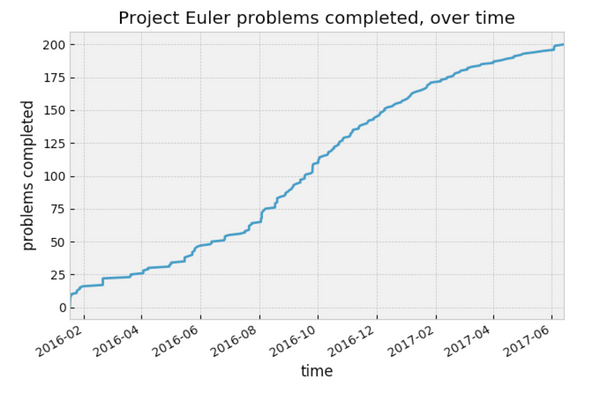There is also the concept of the the "difficulty" of a problem, provided by the website itself as a measure of how often participants get the answer wrong. Here is the cumulative difficulty:Conclusion

I'm not finished with Project Euler yet - there are way to many problems I spent time on but didn't solve and they are just itching me. However, I am taking a break for a while so I can focus on some other projects. After all, solving 200 problems was technically my lifetime goal.

If a person asked me "is Project Euler worth the time?" - I wouldn't immediately say yes. You do need lots of time, and it really is a personal thing (there is a leaderboard, but it's not nearly as public as, say, StackOverflow's leaderboards). You also need to think about the opportunity cost - will solving these problems further your career or research? Personally, I feel that Project Euler rounded out my understanding of computer science and mathematics, and not so heavily loaded on statistics and Python applied to statistics.

]]>
https://dataorigami.net/blogs/napkin-folding/search-through-distributed-datasets-the-modbinary-search 2017-03-04T08:42:00-05:00 2018-04-07T23:15:23-04:00 Searching through distributed datasets: The Mod-Binary Search Cameron Davidson-Pilon On a not-too-unusual day, one of my Spark jobs failed in production. Typically this means there was a row of bad data that entered into the job. As I’m one to write regression tests, this “type” of bad had likely never been seen before, so I needed to inspect the individual offending row (or rows). Typically debug steps include:

1. Manually inspecting all the recent data, either by hand or on a local machine.
2. The failed job might print the offending row in the logs.
3. The failed job might print the stacktrace of the failure, giving a clue as to what the original row looked like.

My problem was not as easy as this:

1. The data is too big to manually inspect, and would not all fit on a single machine to inspect.
2. Nope, unfortunately this is not a feature in Spark logs.
3. Also nope, not in Spark logs. Using Spark DataFrames, all the code is auto-generated at a lower layer anyways, so it returning LOC wouldn’t give me much.

Essentially, all the information I had was the following: my job threw a NullPointerException somewhere when it tried to process this new dataset:

org.apache.spark.SparkException: Job aborted due to stage failure: Task 533 in stage 1.0 failed 4 times, most recent failure: Lost task 533.3 in stage 1.0. java.lang.NullPointerException
Driver stacktrace:
at org.apache.spark.scheduler.DAGScheduler.org$apache$spark$scheduler$DAGScheduler$$failJobAndIndependentStages(DAGScheduler.scala:1454) at org.apache.spark.scheduler.DAGScheduler$$anonfun$abortStage$1.apply(DAGScheduler.scala:1442)
at org.apache.spark.scheduler.DAGScheduleranonfun$abortStage$1.apply(DAGScheduler.scala:1441)
...

But I still needed to finding this offending row. The idea of a binary search over the dataset appealed to me, but the data was distributed: there was no sense of an “order” to it, that is, I had no concept of a middle value to split the data on. The next idea I thought of was using modulus. Suppose each row had an integer 1,2,3,… associated with it, then I could split the data modulo 2 and test either side, 0 or 1, for a failure. Suppose that the 1s-half contained the fai